# JP3776307B2 - Nonvolatile memory analog voltage writing circuit - Google Patents

## Info

Publication number
JP3776307B2
JP3776307B2 JP2000364603A JP2000364603A JP3776307B2 JP 3776307 B2 JP3776307 B2 JP 3776307B2 JP 2000364603 A JP2000364603 A JP 2000364603A JP 2000364603 A JP2000364603 A JP 2000364603A JP 3776307 B2 JP3776307 B2 JP 3776307B2
Authority
JP
Japan
Prior art keywords
memory cell
write
voltage
circuit
source
Prior art date
Legal status (The legal status is an assumption and is not a legal conclusion. Google has not performed a legal analysis and makes no representation as to the accuracy of the status listed.)
Expired - Fee Related
Application number
JP2000364603A
Other languages
Japanese (ja)
Other versions
JP2002015590A (en
Inventor

Original Assignee

Priority date (The priority date is an assumption and is not a legal conclusion. Google has not performed a legal analysis and makes no representation as to the accuracy of the date listed.)
Filing date
Publication date
Priority to JP2000-131531 priority Critical
Priority to JP2000131531 priority
Application filed by 沖電気工業株式会社 filed Critical 沖電気工業株式会社
Priority to JP2000364603A priority patent/JP3776307B2/en
Publication of JP2002015590A publication Critical patent/JP2002015590A/en
Application granted granted Critical
Publication of JP3776307B2 publication Critical patent/JP3776307B2/en
Application status is Expired - Fee Related legal-status Critical
Anticipated expiration legal-status Critical

## Images

••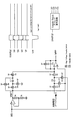•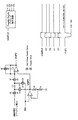•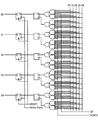•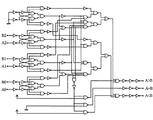•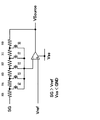•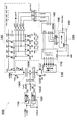•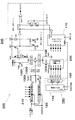•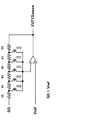•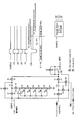•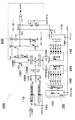•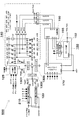•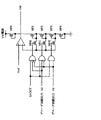•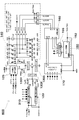•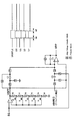•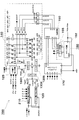•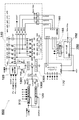•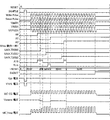•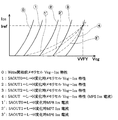•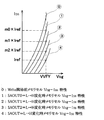•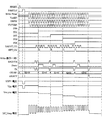•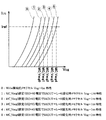•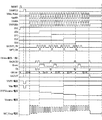•## Classifications

• GPHYSICS
• G11INFORMATION STORAGE
• G11CSTATIC STORES
• G11C11/00Digital stores characterised by the use of particular electric or magnetic storage elements; Storage elements therefor
• G11C11/56Digital stores characterised by the use of particular electric or magnetic storage elements; Storage elements therefor using storage elements with more than two stable states represented by steps, e.g. of voltage, current, phase, frequency
• G11C11/5621Digital stores characterised by the use of particular electric or magnetic storage elements; Storage elements therefor using storage elements with more than two stable states represented by steps, e.g. of voltage, current, phase, frequency using charge storage in a floating gate
• GPHYSICS
• G11INFORMATION STORAGE
• G11CSTATIC STORES
• G11C11/00Digital stores characterised by the use of particular electric or magnetic storage elements; Storage elements therefor
• G11C11/56Digital stores characterised by the use of particular electric or magnetic storage elements; Storage elements therefor using storage elements with more than two stable states represented by steps, e.g. of voltage, current, phase, frequency
• G11C11/5621Digital stores characterised by the use of particular electric or magnetic storage elements; Storage elements therefor using storage elements with more than two stable states represented by steps, e.g. of voltage, current, phase, frequency using charge storage in a floating gate
• G11C11/5628Programming or writing circuits; Data input circuits
• GPHYSICS
• G11INFORMATION STORAGE
• G11CSTATIC STORES
• G11C16/02Erasable programmable read-only memories electrically programmable
• G11C16/06Auxiliary circuits, e.g. for writing into memory
• G11C16/10Programming or data input circuits
• G11C16/12Programming voltage switching circuits
• GPHYSICS
• G11INFORMATION STORAGE
• G11CSTATIC STORES
• G11C27/00Electric analogue stores, e.g. for storing instantaneous values
• G11C27/005Electric analogue stores, e.g. for storing instantaneous values with non-volatile charge storage, e.g. on floating gate or MNOS
• GPHYSICS
• G11INFORMATION STORAGE
• G11CSTATIC STORES
• G11C16/02Erasable programmable read-only memories electrically programmable
• G11C16/06Auxiliary circuits, e.g. for writing into memory
• G11C16/30Power supply circuits
• GPHYSICS
• G11INFORMATION STORAGE
• G11CSTATIC STORES
• G11C19/00Digital stores in which the information is moved stepwise, e.g. shift register stack stores, push-down stores
• GPHYSICS
• G11INFORMATION STORAGE
• G11CSTATIC STORES
• G11C2211/00Indexing scheme relating to digital stores characterized by the use of particular electric or magnetic storage elements; Storage elements therefor
• G11C2211/56Indexing scheme relating to G11C11/56 and sub-groups for features not covered by these groups
• G11C2211/562Multilevel memory programming aspects
• G11C2211/5621Multilevel programming verification
• GPHYSICS
• G11INFORMATION STORAGE
• G11CSTATIC STORES
• G11C2211/00Indexing scheme relating to digital stores characterized by the use of particular electric or magnetic storage elements; Storage elements therefor
• G11C2211/56Indexing scheme relating to G11C11/56 and sub-groups for features not covered by these groups
• G11C2211/564Miscellaneous aspects
• G11C2211/5644Multilevel memory comprising counting devices
• GPHYSICS
• G11INFORMATION STORAGE
• G11CSTATIC STORES
• G11C2211/00Indexing scheme relating to digital stores characterized by the use of particular electric or magnetic storage elements; Storage elements therefor
• G11C2211/56Indexing scheme relating to G11C11/56 and sub-groups for features not covered by these groups
• G11C2211/564Miscellaneous aspects
• G11C2211/5645Multilevel memory with current-mirror arrangements
• GPHYSICS
• G11INFORMATION STORAGE
• G11CSTATIC STORES
• G11C7/00Arrangements for writing information into, or reading information out from, a digital store
• G11C7/06Sense amplifiers; Associated circuits, e.g. timing or triggering circuits
• GPHYSICS
• G11INFORMATION STORAGE
• G11CSTATIC STORES
• G11C8/00Arrangements for selecting an address in a digital store
• G11C8/04Arrangements for selecting an address in a digital store using a sequential addressing device, e.g. shift register, counter

## Description


BACKGROUND OF THE INVENTION
The present invention relates to a technique for storing an analog voltage in an electrically erasable non-volatile memory, and compensates for a write characteristic variation between memory cells and a write characteristic deterioration due to endurance, and between memory cells due to a write characteristic variation. The present invention relates to a circuit for reducing variation in write analog voltage and improving the endurance life.

[Prior art]
Literature name, USP-5,638,320 (date of Patent, Jun. 10, 1997)
In the above document, a method of storing an analog charge amount in a non-volatile memory, its circuit block, and an explanatory diagram are described in FIG. 16 line 6 to 18 Page 19 line and Sheet 29 of 63 FIGURE 12 a to Sheet 40 of 63 FIGURE 15 h. The method of storing the analog charge amount in the nonvolatile memory in this document is as follows.

The threshold voltage of the non-volatile memory cell becomes saturated with a decrease in threshold change per unit time as the write time increases, and the write saturation threshold voltage is proportional to the control voltage at the time of writing. Short writing is repeated in proportion to the analog voltage to which the control voltage at the time of writing is written (write operation), and the threshold voltage of the memory cell is read and compared with the analog voltage to be written during the write (verify operation). This write / verify operation is repeated, and the write operation is terminated when the threshold voltage of the read memory cell reaches the analog voltage to be written.

Further, in this document, as an improvement method for shortening the write analog voltage accuracy and the analog write time, a method of changing the write control voltage and the write / verify time during the write / verify repetitive operation instead of making them uniform (from FIGURE 15a of Sheet 33 of 63). FIG. 15h) of Sheet 40 of 63 is disclosed.

[Problems to be solved by the invention]
However, the method of changing the write control voltage and the write / verify time during the write / verify repetitive operation disclosed in the above-mentioned document instead of making them uniform (FIGURE 15a of Sheet 33 of 63 to FIG. 15 h of Sheet 40 of 63) is the initial stage of writing start. By increasing the initial write speed by setting the control voltage at the time of writing higher than the proportional voltage of the original write analog voltage or by shortening the write pulse width at the beginning of writing to increase the write injection efficiency Even if the initial write speed is slow or the endurance deterioration causes the write speed to slow down, complete the analog voltage write operation within a specified time to improve the write analog voltage accuracy. Although it is an, in this way there is a risk that the write analog voltage accuracy early cell of initial write speed will reach the analog voltage written in during the initial write operation is rough.

The only way to prevent a cell with a fast initial write speed from reaching the analog voltage written during the initial write operation is to reduce the initial write speed. As described above, the improvement method for reducing the write analog voltage accuracy and the analog write time disclosed in this document does not take measures against the cell having a high initial write speed, and the write analog voltage is limited by the restriction of the cell having the high initial write speed. There was a problem that voltage accuracy and analog writing time reduction could not be improved sufficiently.

The present invention has been made in view of the above-mentioned problems of the conventional nonvolatile memory analog voltage writing circuit, and an object of the present invention is to improve the accuracy of the writing voltage and shorten the writing time. It is a novel and improved non-volatile memory analog voltage writing circuit.

[Means for Solving the Problems]
In order to solve the above-described problem, according to claim 1, in a circuit for storing an analog voltage in an electrically erasable / erasable nonvolatile memory, an applied analog voltage value is applied to a control gate of the memory cell at the time of memory cell writing. A write voltage generation circuit (110) for generating a write voltage (Vpp) to be generated, and a verify voltage generation for generating a verify voltage (VVFY) to be applied to the control gate of the memory cell at the time of memory cell verification from the input analog voltage value A circuit (120) and a source voltage (VSource) to be applied to the source of the memory cell when the memory cell is written are generated, and the output source voltage is changed by changing the gain of the inverting amplifier by the input of the control signals (S0 to S4). Source voltage generator (135) and memo A multi-level sense amplifier (140) for comparing the current mirror specific current of the memory cell drain current with the comparison reference current (Iref) at the time of cell verification, and a counter-decoder circuit (150) for outputting a signal at a predetermined number of write signal pulses. A comparison circuit (160) for comparing the output of the multi-level sense amplifier and the output of the counter-decoder circuit, an up / down shift register circuit (170) for shifting the level of the control signal according to the comparison result of the comparison circuit, A non-volatile memory analog voltage writing circuit (100) is provided.

According to such a configuration, the decoder circuit output that changes from L to H in a predetermined order (A0 → A1 → A2) according to the write time, and the output in a predetermined order (SAOUT0 → SAOUT1 → SAOUT2) due to the memory cell threshold voltage rise. The comparison circuit compares the output of the multi-level sense amplifier circuit that changes from L to H with the comparison circuit, and the source voltage applied to the source of the memory cell can be changed according to the result.

Therefore, a cell with a slow write speed can increase the voltage between the source and control gates of the memory cell at the time of write, and a cell with a fast write speed can reduce the voltage between the source and control gate of the memory cell at the time of write. The memory cell write operation is possible while searching for the voltage condition between the source and control gates of the write memory cell in accordance with the write characteristics of the selected memory cell.

According to a second aspect of the present invention, in a circuit for storing an analog voltage in an electrically writable / erasable non-volatile memory, a write voltage (Vpp) applied to the control gate of the memory cell when the memory cell is written from the input analog voltage value A write voltage generation circuit (110) for generating a verification voltage generation circuit (120) for generating a verification voltage (VVFY) to be applied to the control gate of the memory cell at the time of memory cell verification from the input analog voltage value; A source voltage generation circuit (135) that generates a source voltage (VSource) to be applied to the source of the memory cell at the time of writing to the memory cell and changes the output source voltage by changing the gain of the inverting amplifier by the input of the control signals (S0 to S4). ) And the memory cell verify A multilevel sense amplifier (140) for comparing the resell drain current with the current mirror ratio current of the comparison reference current (Iref), a counter-decoder circuit (150) for outputting a signal at a predetermined number of write signal pulses, and the multilevel A comparison circuit (160) for comparing the output of the sense amplifier and the output of the counter-decoder circuit; and an up / down shift register circuit (170) for shifting the level of the control signal according to the comparison result of the comparison circuit. A non-volatile memory analog voltage write circuit (200) is provided.

According to such a configuration, the decoder circuit output that changes from L to H in a predetermined order (A0 → A1 → A2) according to the write time, and the output in a predetermined order (SAOUT0 → SAOUT1 → The output from the multi-level sense amplifier circuit that changes from L to H in SAOUT2) is compared by the comparison circuit, and the source voltage applied to the source of the memory cell can be changed according to the result.

Therefore, a cell with a slow write speed can increase the voltage between the source and control gates of the memory cell at the time of write, and a cell with a fast write speed can reduce the voltage between the source and control gate of the memory cell at the time of write. The memory cell write operation is possible while searching for the voltage condition between the source and control gates of the write memory cell in accordance with the write characteristics of the selected memory cell.

According to a third aspect of the present invention, in a circuit for storing an analog voltage in an electrically writable / erasable non-volatile memory, a write voltage (Vpp) applied to the control gate of the memory cell when the memory cell is written from the input analog voltage value Level shift output write voltage after sample and hold by increasing the voltage of the capacitor ratio amplification AMP negative terminal and the symmetric electrode of the charge distribution capacitor (C1) by the input of the first control signal (S0 to S4) A write voltage generation circuit (210) that changes the voltage, a verify voltage generation circuit (120) that generates a verify voltage (VVFY) to be applied to the control gate of the memory cell at the time of memory cell verification from the input analog voltage value, and a memory Memory cell source during cell verification A source voltage generation circuit (235) for generating a verification source voltage (VVFYSource) to be applied to the first and second output signals SX0 to SX4, and changing the output verification source voltage by changing the gain of the inverting amplifier. From the sense amplifier (240) that compares the memory cell drain current with the comparison reference current (Iref) at the time of cell verification, the counter-decoder circuit that outputs a signal at a predetermined number of write signal pulses, and the sense amplifier output (SAOUT0), A non-volatile memory analog voltage writing circuit (300) is provided, comprising an up / down shift register circuit (170) for shifting the level of the first control signal (S0 to S4).

According to such a configuration, the decoder circuit output that changes from L to H in a predetermined order (for example, SX0 → SX1 → SX2 → SX3 → SX4) according to the write time, and the verify source voltage by the output (SX0 to SX4) (VVFYSource) is applied to the source of the selected memory cell with the output voltage of the source voltage generation circuit that changes and drops to increase the voltage between the memory cell source and the control gate at the time of verifying, and the memory cell according to the sense amplifier output result The write voltage (Vpp) at the time of writing can be changed.

For this reason, a cell with a slow write speed can increase the write voltage, while a cell with a fast write speed can decrease the write voltage, while searching for a write voltage condition that matches the write characteristics of the selected memory cell. Memory cell write operation is possible.

According to a fourth aspect of the present invention, in a circuit for storing an analog voltage in an electrically writable / erasable nonvolatile memory, a write voltage (Vpp) applied to the control gate of the memory cell when the memory cell is written from the input analog voltage value A write voltage generation circuit (110) for generating a verification voltage generation circuit (120) for generating a verification voltage (VVFY) to be applied to the control gate of the memory cell at the time of memory cell verification from the input analog voltage value; A source voltage generation circuit that generates a source voltage (VSource) to be applied to the source of a memory cell when writing to the memory cell, and changes the output source voltage by changing the gain of the inverting amplifier according to the input of the first control signal (S0 to S4). (135) and memory cell verify A source voltage generation circuit (235) that generates a verify source voltage (VVFYSource) to be applied to the source of the memory cell and changes the output verify source voltage by changing the gain of the inverting amplifier according to the input of the second control signals (SX0 to SX4). ), A sense amplifier (240) that compares the memory cell drain current with the comparison reference current at the time of memory cell verification, a counter-decoder circuit (150) that outputs a signal at a predetermined number of write signal pulses, and the sense amplifier output A non-volatile memory analog voltage writing circuit (400) is provided, comprising an up / down shift register circuit (170) for shifting the level of the first control signal.

According to this configuration, the verify source voltage is determined by the decoder circuit output that changes from L to H in a predetermined order (SX0 → SX1 → SX2 → SX3 → SX4) and its output (SX0 to SX4) according to the number of write pulses (write time). Is applied to the source of the selected memory cell to increase the voltage between the memory cell source and the control gate at the time of verification, and the memory cell is written at the time of writing to the memory cell according to the sense amplifier output result. The source voltage applied to the source can be changed.

Therefore, a cell with a slow write speed can increase the voltage between the source and control gates of the memory cell at the time of write, and a cell with a fast write speed can reduce the voltage between the source and control gate of the memory cell at the time of write. The memory cell write operation is possible while searching for the voltage condition between the source and control gates of the write memory cell in accordance with the write characteristics of the selected memory cell.

According to a fifth aspect of the present invention, in a circuit for storing an analog voltage in an electrically writable / erasable non-volatile memory, a write voltage (Vpp) applied to a control gate of the memory cell at the time of memory cell write from the input analog voltage value Level shift output write voltage after sample and hold by increasing the voltage of the capacitor ratio amplification AMP negative terminal and the symmetric electrode of the charge distribution capacitor (C1) by the input of the first control signal (S0 to S4) A write voltage generation circuit (210) that changes the voltage, a verify voltage generation circuit (120) that generates a verify voltage (VVFY) to be applied to the control gate of the memory cell at the time of memory cell verification from the input analog voltage value, and a memory Write memory cell when writing cell A write memory cell drain voltage generation circuit (VW voltage generation circuit 125) that generates a rain voltage (VW voltage) and changes a gain of a non-inverting amplifier to change a write memory cell drain voltage, and a memory cell drain during memory cell verification A multilevel sense amplifier (140) for comparing the current current ratio of the current to a comparison reference current (Iref); a counter-decoder circuit (150) for outputting a signal at a predetermined number of write signal pulses; and the multilevel sense amplifier A comparison circuit (160) for comparing the output with the counter-decoder circuit output; and an up / down shift register circuit (170) for shifting the level of the first control signal output according to the comparison result of the comparison circuit. Nonvolatile memory analog voltage writing circuit 500) is provided.

According to this configuration, the decoder circuit output changes from L to H in a predetermined order (A0 → A1 → A2) according to the number of write pulses (write time) and the output is in a predetermined order (SAOUT0) due to the rise in the memory cell threshold value Vt. The output from the multi-level sense amplifier circuit that changes from L → H in (SAOUT1 → SAOUT2) is compared by the comparison circuit, and the memory cell write voltage can be changed according to the result.

For this reason, a cell with a slow write speed can increase the write voltage, while a cell with a fast write speed can decrease the write voltage, while searching for a write voltage condition that matches the write characteristics of the selected memory cell. A memory cell write operation is possible. For cells with extremely slow write speed, the write memory cell drain voltage of the memory cell at the time of write can be increased to increase the write speed.

According to a sixth aspect of the present invention, in a circuit for storing an analog voltage in an electrically writable / erasable non-volatile memory, a write voltage (Vpp) applied to the control gate of the memory cell when the memory cell is written from the input analog voltage value ) Is generated, and a write voltage is generated to change the level shift output write voltage after the sample hold by increasing the capacitor ratio amplification AMP positive terminal voltage of the charge distribution capacitor (C1) by the input of the control signals (S0 to S4) A circuit (310), a verify voltage generation circuit (120) for generating a verify voltage (VVFY) to be applied to the control gate of the memory cell at the time of memory cell verification from the input analog voltage value, and a write memory cell at the time of memory cell writing Drain voltage (V Voltage) and a write memory cell drain voltage generation circuit (VW voltage generation circuit 125) that changes the write memory cell drain voltage by changing the gain of the non-inverting amplifier, and a current mirror drain of the memory cell drain current during memory cell verification A multilevel sense amplifier (140) for comparing a specific current with a comparison reference current; a counter-decoder circuit (150) for outputting a signal for a predetermined number of write signal pulses; the multilevel sense amplifier output; and the counter-decoder circuit A non-volatile memory analog comprising: a comparison circuit (160) for comparing the output; and an up / down shift register circuit (170) for shifting the level of the control signal according to a comparison result of the comparison circuit. A voltage writing circuit (600) is provided.

According to this configuration, the decoder circuit output changes from L to H in a predetermined order (A0 → A1 → A2) according to the number of write pulses (write time) and the output is in a predetermined order (SAOUT0) due to the rise in the memory cell threshold value Vt. The output from the multi-level sense amplifier circuit that changes from L → H in (SAOUT1 → SAOUT2) is compared by the comparison circuit, and the memory cell write voltage can be changed according to the result.

For this reason, a cell with a slow write speed can be operated to increase the write voltage during write, and a cell with a fast write speed can be decreased to reduce the write voltage during write. The memory cell write operation can be performed while searching. For a cell with extremely slow write speed, the write applied speed can be increased by increasing the drain applied VW voltage of the memory cell at the time of write.

According to a seventh aspect of the present invention, in a circuit for storing an analog voltage in an electrically writable / erasable non-volatile memory, a write voltage (Vpp) applied to a control gate of the memory cell at the time of memory cell write from the input analog voltage value ), And a voltage generation circuit that changes the level shift output write voltage after the sample hold by increasing the voltage of the capacitor ratio amplification AMP negative terminal and the symmetrical electrode of the charge distribution capacitor (C1) by the input of the control signal ( 210), a verify voltage generation circuit (120) for generating a verify voltage (VVFY) to be applied to the control gate of the memory cell when verifying the memory cell from the input analog voltage value, and a write memory cell drain voltage when writing to the memory cell (VW voltage) And a write memory cell drain voltage generation circuit (VW voltage generation circuit 125) that changes the write memory cell drain voltage by changing the gain of the non-inverting amplifier, and a comparison reference current (Iref ), A counter-decoder circuit (150) for outputting a signal for a predetermined number of write signal pulses, the multi-level sense amplifier output, and the counter-decoder circuit. A non-volatile memory analog comprising: a comparison circuit (160) for comparing the output; and an up / down shift register circuit (170) for shifting the level of the control signal according to a comparison result of the comparison circuit. A voltage writing circuit (700) is provided.

According to such a configuration, the decoder circuit output changes from L to H in a predetermined order (A0 → A1 → A2) according to the number of write pulses (write time), and the output is in a predetermined order (SA0UT0) due to the rise of the memory cell threshold value Vt. The output of the multi-level sense amplifier circuit that changes from L to H in (SA0UT1 → SAOUT2) is compared by the comparison circuit, and the memory cell write voltage can be changed according to the result.

For this reason, a cell with a slow write speed can be operated to increase the write voltage during write, and a cell with a fast write speed can be decreased to reduce the write voltage during write. The memory cell write operation can be performed while searching. For a cell with extremely slow write speed, the write applied speed can be increased by increasing the drain applied VW voltage of the memory cell at the time of write.

According to the eighth aspect of the present invention, in the circuit for storing an analog voltage in an electrically writable / erasable nonvolatile memory, the write voltage (Vpp) applied to the control gate of the memory cell when the memory cell is written from the input analog voltage value And a write voltage generation circuit (310) for changing the level shift output write voltage after the sample hold by increasing the capacitor ratio amplification AMP positive terminal voltage by the input of the control signal (S0 to S4), and an input A verify voltage generation circuit (120) for generating a verify voltage (VVFY) to be applied to the control gate of the memory cell at the time of memory cell verification from the analog voltage value, and a write memory cell drain voltage (VW voltage) at the time of memory cell writing Generated, non-inverted increase A write memory cell drain voltage generation circuit (VW voltage generation circuit 125) that changes the gain of the write circuit to change the write memory cell drain voltage, and compares the memory cell drain current with the current reference current of the comparison reference current when the memory cell is verified A multi-level sense amplifier (240), a counter-decoder circuit (150) for outputting a signal at a predetermined number of write signal pulses, and a comparison circuit for comparing the multi-level sense amplifier output with the counter-decoder circuit output ( 160) and an up / down shift register circuit (170) for shifting the level of the control signal according to the comparison result of the comparison circuit, a nonvolatile memory analog voltage writing circuit (800) is provided. Is done.

According to this configuration, the decoder circuit output changes from L to H in a predetermined order (A0 → A1 → A2) according to the number of write pulses (write time) and the output is in a predetermined order (SAOUT0) due to the rise in the memory cell threshold value Vt. The output from the multi-level sense amplifier circuit that changes from L → H in (SAOUT1 → SAOUT2) is compared by the comparison circuit, and the memory cell write voltage can be changed according to the result.

For this reason, a cell with a slow write speed can be operated to increase the write voltage during write, and a cell with a fast write speed can be decreased to reduce the write voltage during write. The memory cell write operation can be performed while searching. For a cell with extremely slow write speed, the write applied speed can be increased by increasing the drain applied VW voltage of the memory cell at the time of write.

DETAILED DESCRIPTION OF THE INVENTION
Exemplary embodiments of a nonvolatile memory analog voltage writing circuit (hereinafter simply referred to as “writing circuit”) according to the present invention will be described below in detail with reference to the accompanying drawings. In the accompanying drawings, FIGS. 1 to 17 show circuit diagrams of the respective embodiments, and FIGS. 18 to 24 show timing diagrams and the like common to the respective embodiments. Further, in the present specification and the drawings, the same reference numerals are given to constituent elements having substantially the same functional configuration, and redundant description is omitted. Further, the high level potential is represented as “H”, and the low level potential is represented as “L”.

(First embodiment)
FIG. 1 shows a circuit diagram of a first embodiment of the present invention. The write circuit 100 includes a write voltage generation circuit 110 that generates a write voltage Vpp to be applied to the control gate of the memory cell when the memory cell is written from the input analog voltage value, and a memory at the time of verifying the memory cell from the input analog voltage value. A verify voltage generation circuit 120 for generating a verify voltage VVFY to be applied to the control gate of the cell is provided.

Further, the write circuit 100 includes an X decoder 130X / Y decoder 130Y / source decoder 130S for selecting a write memory cell, a transfer gate MP1 for conducting the write voltage Vpp to the X decoder 130X at the time of memory cell write, and at the time of memory cell verification. A transfer gate MP2 that conducts the verify voltage VVFY to the X decoder 130X, a transfer gate MP3 that conducts the write memory cell drain voltage VW to the Y decoder 130Y during memory cell writing, and a source voltage VSource to the source decoder 130S during memory cell writing. A transfer gate MN8 for conducting, and a transfer gate MN7 for conducting the GND voltage to the source decoder 130S during memory cell verification, A source voltage generation circuit 135 that generates a source voltage VSource to be applied to the source of the memory cell when writing to the memory cell, and a source follower NMOS transistor that causes the Y decoder 130Y to conduct a drain voltage that prevents read disturb of the memory cell when verifying the memory cell MN1 is provided.

Further, the write circuit 100 includes MP4 to MP8, MN2 to MN5, INV0 to INV3 constituting a multilevel sense amplifier 140 that compares the memory cell drain current with the comparison reference current Iref at the time of memory cell verification, and a predetermined write signal pulse. A counter-decoder circuit 150 that outputs signals to the number, a comparison circuit 160 that compares the multi-level sense amplifier 140 outputs SAOUT0 to SAOUT2 with the counter-decoder circuit 150 outputs A0, A1, and A2, and outputs S0 to S0 according to the comparison result. An up / down shift register circuit 170 for shifting S4 in both MSB / LSB directions is provided. Up-down shift register circuit 170 outputs S0 to S4 are input to source voltage generation circuit 135.
The write circuit 100 is configured as described above.

FIG. 2 shows a circuit diagram of the write voltage generation circuit 110. The write voltage generation circuit 110 is a sample-and-hold level shift circuit, and the shift amount (gain) is a constant value.
FIG. 3 is a circuit diagram of the verify voltage generation circuit 120. The verify voltage generation circuit 120 is also a sample and hold level shift circuit, but its shift amount (gain) is a constant value.
FIG. 4 shows a circuit diagram of the up / down shift register circuit 170.
FIG. 5 shows a circuit diagram of the comparison circuit 160.
FIG. 6 shows a circuit diagram of the source voltage generation circuit 135. The source voltage generation circuit 135 is composed of an inverting amplifier, and is characterized in that the output source voltage VSource can be changed by changing the gain of the inverting amplifier by S0 to S4 inputs.

FIG. 18 shows a timing chart of the first embodiment. After the erase target memory cell is selected by the X decoder 130X / Y decoder 130Y / source decoder 130S, the counter circuit 152 and the up / down shift register circuit 170 are first reset by a reset pulse RESET. The reset state of the up / down shift register circuit 170 is S2 = H.

The input voltage is sampled and held and level-shifted by the write voltage generator 110 and the verify voltage generator 120 in response to the signal SAMPLE, thereby generating the write voltage Vpp and the verify voltage VVFY. The relationship between the write voltage Vpp and verify voltage VVFY at that time and the input analog voltage value AINREC is as follows.
Vpp = C2 / C3 × (AINREC−SG) + (C1 / C3 + 1) × VSFT
VVFY = C1 / C2 × (AINREC−SG) + VSFT

When the write pulse and the clock signals of the enable signals VppEN, VWEN, and VVFYEN are given to the counter circuit 152 and the up / down shift register circuits 170, MP1, MP2, MP3, MN7, and MN8, the write / verify operation is started.

FIG. 19 shows the memory cell Vcg-Ids characteristics and SAOUT0 to SAOUT2 output relationship during writing in the circuit operation of the first embodiment. As the memory cell threshold value Vt rises due to repeated write / verify operations, the Vcg-Ids characteristic of the memory cell changes from 0 → ▲ 1 ▼ → ▲ 2 ▼ → ▲ 3 ▼ → ▲ 4 ▼ in FIG. go. In the first embodiment, the memory cell drain current at the time of memory cell verification is changed by the current mirror circuit composed of MP4 to MP8 and compared with the comparison reference current Iref.

Since MP4: MP5: MP6: MP7: MP8 = 1: 1/1 / m2: 1 / m1: 1 / m0 (1> 1 / m2> 1 / m1> 1 / m0), the memory cell threshold value is set. Vt rise 0 → ▲ 1 ▼ → ▲ 2 ▼ → ▲ 3 ▼ → ④4 In response to the change, the sense amplifier inverter inverts L → H in the order of INV0 to INV3, and the multi-level sense amplifier 140 output is also SAOUT0 → SAOUT1 → It changes from L to H in the order of SAOUT2. The speed of increase of the memory cell threshold value Vt is determined by the memory cell characteristics and the source-control voltage during writing.

At the start of writing, outputs A0 to A2 of the decoder circuit 155 are all set to L. After that, it changes from L to H in the order of A0 → A1 → A2 according to the number of write pulses (write time) after the start of writing.

At the start of writing,
Write voltage Vpp (constant value), Vpp = C2 / C3 × (AINREC−SG) + (C1 / C3 + 1) × VSFT
Initial setting source voltage VSource, VSource = (R0 + R1 + R2) / (R3 + R4 + R5) × (SG−Vref) + Vref
At the start of writing,
Write voltage Vpp (constant value) −initial setting source voltage VSource
= [C2 / C3 * (AINREC-SG) + (C1 / C3 + 1) * VSFT. − [− (R0 + R1 + R2) / (R3 + R4 + R5) × (SG−Vref) + Vref
However, the voltage becomes the source-control gate voltage of the selected memory cell at the time of writing, the selected memory cell is written, and the memory cell threshold voltage Vt rises.

Then, the multi-level sense amplifier 140 outputs SAOUT0 to SAOUT2 and the decoder circuit 155 outputs A0 to A2 are compared by the comparison circuit 160, and the up / down shift register circuit 170 output is changed and the source voltage VSource is changed as a result. ing.

If SAOUT0 changes from L to H before A0 changes from L to H, the output A <B of the comparison circuit 160 changes from L to H, and S2 = H of the up / down shift register circuit 170 becomes S1 =. Shift to H,
The source voltage VSource is
VSource = − (R0 + R1) / (R2 + R3 + R4 + R5) × (SG−Vref) + Vref
The voltage between the source and the control gate of the selected memory cell is decreased during writing.

Conversely, when A0 changes from L to H before SAOUT0 changes from L to H, the output A> B of the comparison circuit 160 changes from L to H and S2 of the up / down shift register circuit 170 changes. = H shifts to S3 = H, and the source voltage VSource is
VSource = − (R0 + R1 + R2 + R3) / (R4 + R5) × (SG−Vref) + Vref
The voltage between the source and control gate of the selected memory cell increases during writing.

The timing diagram of FIG. 18 shows the case where A0 changes from L to H before the latter SAOUT0 changes from L to H.

Similarly, when the output of the decoder circuit 155 is changed from L → H in the order of A1 → A2 in accordance with the number of write pulses (write time), the multilevel sense amplifier 140 outputs SAOUT0 to SAOUT2 and the decoder circuit at the time of verifying The 155 outputs A0 to A2 are compared by the comparison circuit 160, and the output of the up / down shift register circuit 170 is changed according to the result, and the source voltage VSource is changed.

Decoder circuit 155 outputs A0 to A2 L → H change write pulse number (write time) and power level mirror circuit MP4: MP5: MP6: MP7: MP8 = 1: 1/1 / m2: 1 / m1: 1 / m0 (1> 1 / m2> 1 / m1> 1 / m0) If the ratio coefficient is matched with the type of memory cell used in the write time-write Vt characteristic of the memory cell, the write speed Slow cells can increase the voltage between the source and control gates of the memory cell at the time of writing, and conversely, cells with a high writing speed can operate to decrease the voltage between the source and control gates of the memory cell at the time of writing. Write conditions according to the characteristics (Voltage between source and control gate of memory cell during write To continue the memory cell write operation while looking for.

Then, when the memory cell drain current becomes equal to or lower than the comparison reference current Iref, SAOUT is output H, and the write operation of the selected memory cell is completed.

As described above, in the first embodiment, the output is SAOUT0 due to the output of the decoder circuit 155 changing from L0 to H in the order of A0 → A1 → A2 according to the number of write pulses (write time) and the memory cell threshold value Vt rising. Since the comparison circuit 160 compares the output of the multi-level sense amplifier 140 changing from L → H in the order of SAOUT1 → SAOUT2 and the memory cell write source voltage VSource is changed according to the result, the write speed is increased. Slow cells can increase the voltage between the source and control gates of the memory cell at the time of writing, and conversely, cells with a high writing speed can decrease the voltage between the source and control gates of the memory cell at the time of writing. Write conditions that match the characteristics (memory during writing Le sources - it is possible to perform the memory cell write operation of looking for the inter-control gate voltage).

Therefore, it is possible to terminate the analog write operation without degrading the write analog voltage accuracy within a predetermined time even in a cell having a low initial write speed or a cell having a low write speed due to endurance degradation. Furthermore, the final analog voltage can be written with memory cell write Vt saturation even in a cell with a fast initial write speed, and variations in analog voltage accuracy can be improved.

In the first embodiment, the source voltage generation circuit 135 can set the reference voltage Vref and the gain resistances R0 to R5 of the inverting amplification AMP so that the output source voltage VSource is equal to or higher than GNP. When a non-selected erase state memory cell on the same bit line as the write selected memory cell includes an over erase state memory cell, the source voltage VSource of the over erase state memory cell can be made to be equal to or higher than GND. It is possible to prevent Ids leakage in the memory cell, and it is not necessary to take measures against overerased memory cells, such as making the current capability of the VW power supply of the write drain voltage equal to or higher than the write Ids of the write selected memory cell. When the VW power supply is generated by the LIS internal booster circuit, an increase in current consumption can be suppressed.

Conversely, in the source voltage generation circuit 135, the reference voltage Vref and the gain resistances R0 to R5 of the inverting amplification AMP can be set so that the output source voltage VSource is equal to or lower than GND. In that case, the write voltage Vpp (constant value), which is an applied voltage applied to the control gate of the memory cell at the time of writing, can be lowered. In this case, the gate disturb can be reduced because the control gate applied voltage of the non-selected memory cell on the same word line as the write selected memory cell is also lowered.

As described above, in the first embodiment, the source voltage generation circuit 135 output source voltage VSource is set to be equal to or higher than GND for a memory cell in which an overerased cell is likely to occur in accordance with the characteristics of the memory cell in the process used. Thus, the Ids leak of the overerased cell can be prevented, and for the memory cell having a weak gate disturb, the output voltage Vsource of the source voltage generation circuit 135 is set to GND or lower and the write voltage Vpp is lowered to reduce the gate. Disturbance can be prevented.

(Second Embodiment)
FIG. 7 shows a circuit diagram of the second embodiment of the present invention. In the write circuit 200, the capacity ratio of MP4 to MP8 and MN2 to MN5 constituting the multilevel sense amplifier 140 is MP4: MP5: MP6: MP7: MP8 = 1: 1: 1: 1: 1 and MN6: MN2: MN3: MN4: MN5 = 1: 1: m2: m1: m0 (1 <m2 <m1 <m0), which is different from the capacity ratio of MP4 to MP8, MN2 to MN5 of the first embodiment. Except for this, it is the same as the write circuit 100 of the first embodiment.

FIG. 18 shows a timing chart of the second embodiment. The timing chart of the second embodiment is the same as that of the first embodiment, and the basic operation is the same. The difference lies in the circuit operation of the third embodiment shown in FIG. 20 in that the memory cell Vcg-Ids characteristics and SAOUT0 to SAOUT2 output relationship during writing in the circuit operation of the first embodiment shown in FIG. Is the memory cell Vcg-Ids characteristic and the SAOUT0 to SAOUT2 output relationship.

In FIG. 20, the Vcg-Ids characteristic of the memory cell changes from 0 → (1) → (2) → (3) → (4) in FIG. 20 as the memory cell threshold value Vt rises by the repeated write / verify operation. In the third embodiment, the comparison current with the M drain current at the time of memory cell verification is changed to a current by changing the comparison reference current Iref with a current mirror circuit composed of MN2 to MP5. Is a point.

Since MN6: MN2: MN3: MN4: MN5 = 1: 1: m2: m1: m0 (1 <m2 <m1 <m0), the memory cell threshold Vt increases 0 → ▲ 1 ▼ → ▲ 2 ▼ → ▲ In response to the change, the sense amplifier inverter is inverted from L to H in the order of INV0 to INV3, and the output of the multi-level sense amplifier 140 is also changed from L to H in the order of SAOUT0 → SAOUT1 → SAOUT2. The speed of the rise of the cell threshold Vt is the same in that it is determined by the memory cell characteristics and the write voltage Vpp at the time of writing.

Similarly to the first embodiment, in the second embodiment, the output of the decoder circuit 155 and the memory cell threshold value Vt that change from L to H in the order of A0 → A1 → A2 according to the number of write pulses (write time). The comparison circuit 160 compares the output of the multilevel sense amplifier 140 whose output changes from L → H in the order of SAOUT0 → SAOUT1 → SAOUT2 due to the rise, and the memory cell write source voltage VSource is changed according to the result. A cell with a slow write speed can increase the voltage between the source and control gates of the memory cell during writing, while a cell with a fast write speed can be operated to decrease the voltage between the source and control gates of the memory cell during writing. Write conditions that match the write characteristics of the memory cell (write The source of the memory cell - can perform memory cell write operation of looking for the inter-control gate voltage).

Therefore, it is possible to terminate the analog write operation without degrading the write analog voltage accuracy within a predetermined time even in a cell having a low initial write speed or a cell having a low write speed due to endurance degradation. Furthermore, the final analog voltage can be written with memory cell write Vt saturation even in a cell with a fast initial write speed, and variations in analog voltage accuracy can be improved.

In addition, the source voltage generation circuit 135 can set the reference voltage Vref and the gain resistances R0 to R5 of the inverting amplification AMP so that the output source voltage VSource is equal to or higher than GND.

When a non-selected erase state memory cell on the same bit line as the write selected memory cell includes an over erase state memory cell, the source voltage VSource of the over erase state memory cell can be made to be equal to or higher than GND. It is possible to prevent the Ids leak of the memory cell, and it is not necessary to take measures against overerased memory cells such as setting the current capability of the VW power supply of the write drain voltage to be equal to or higher than the write Ids of the write selected memory cell. When the VW power supply is generated by the LIS internal booster circuit, an increase in current consumption can be suppressed.

Conversely, in the source voltage generation circuit 135, the reference voltage Vref and the gain resistances R0 to R5 of the inverting amplification AMP can be set so that the output source voltage VSource is equal to or lower than GND. In that case, the write voltage Vpp (constant value), which is an applied voltage applied to the control gate of the memory cell at the time of writing, can be lowered. In this case, the gate disturb can be reduced because the control gate applied voltage of the non-selected memory cell on the same word line as the write selected memory cell is also lowered.

As described above, the source voltage generation circuit 135 output source voltage VSource is set to be equal to or higher than GND for a memory cell in which an overerased cell is likely to occur in accordance with the characteristics of the memory cell in use process. For memory cells with weak gate disturbance, it is possible to set the source voltage VSource at the source voltage generation circuit 135 to GND or lower to lower the write voltage Vpp to prevent gate disturbance. It is.

Further, in the second embodiment, the multi-level sense amplifier 140 is configured such that the NchMOS current mirror ratio on the comparison reference Iref current source side is MN6: MN2: MN3: MN4: MN5 = 1: 1: m2: m1: m0 (1 <m2 Since it is changed to <m1 <m0), the memory cell threshold value Vt confirmation in the middle of writing INV0 to INV2 is performed with the comparison reference current Iref ′ larger than the comparison reference Iref current. Therefore, the memory cell threshold value Vt can be confirmed in the middle of writing INV0 to INV2 at a higher speed than when the comparison reference current Iref is performed with the comparison reference current Iref. The sense amplifier according to the second embodiment Becomes a high-speed multi-level sense amplifier 140. Therefore, in the second embodiment, the sense amplifier response time of the write memory cell threshold value Vt confirmation time performed in the verify period of the verify repeat operation can be shortened, which is effective when the write pulse frequency is increased. Is.

Since the memory cell threshold value Vt is confirmed with the comparison reference current Iref ′ larger than the comparison reference current Iref during the writing INV0 to INV2, the comparison reference current Iref ′ is applied to the PchMOSs (MP4 to MP8) of the current mirror circuit. It is necessary to adjust to large MN5. Therefore, when the circuit of the second embodiment is configured by a process of μp> μn, there is an advantage that the layout area can be reduced without increasing the dimension of MP4.

(Third embodiment)
FIG. 8 shows a circuit diagram of the third embodiment of the present invention. The write circuit 300 includes a write voltage generation circuit 210 that generates a write voltage Vpp to be applied to the control gate of the memory cell when the memory cell is written from the input analog voltage value, and a memory when the memory cell is verified from the input analog voltage value. A verify voltage generation circuit 120 for generating a verify voltage VVFY to be applied to the control gate of the cell is provided.

Further, the write circuit 300 includes an X decoder 130X / Y decoder 130Y / source decoder 130S for selecting a write memory cell, a transfer gate MP1 for conducting the write voltage Vpp to the X decoder 130X at the time of memory cell write, and at the time of memory cell verification. A transfer gate MP2 for conducting the verify voltage VVFY to the X decoder 130X, a transfer gate MP3 for conducting the write memory cell drain voltage VW to the Y decoder 130Y at the time of memory cell writing, and a GND voltage to the source decoder 130S at the time of memory cell writing. A transfer gate MN5, and a transfer for conducting the verify source voltage VVFYSource to the source decoder 130S during memory cell verification The gate MN4, a source voltage generation circuit 235 that generates a verify source voltage VVFYSource applied to the source of the memory cell at the time of memory cell verification, and a drain voltage that prevents read disturb of the memory cell at the time of memory cell verification are made conductive to the Y decoder 130Y. A source follower NMOS transistor MN1 is provided.

Further, the write circuit 300 includes MP4, MP5, MN2, MN3, and INV0 constituting the sense amplifier 240 that compares the memory cell drain current with the comparison reference current Iref at the time of verifying the memory cell, and signals when a predetermined number of write signal pulses is reached. And an up / down shift register circuit 170 for shifting the outputs S0 to S4 in both MSB / LSB directions from the sense amplifier output SAOUT0. The outputs S0 to S4 of the up / down shift register circuit 170 are input to the write voltage generation circuit 110. The counter-decoder circuit 150 outputs SX0 to SX4 are input to the write voltage generation circuit 110.
The write circuit 300 is configured as described above.

The writing circuit 300 includes a source voltage generating circuit 235 instead of the source voltage generating circuit 135 of the above embodiment. FIG. 9 shows a circuit diagram of the source voltage generation circuit 235. The source voltage generation circuit 235 is composed of an inverting amplifier, and is characterized in that the output verify source voltage VVFYSource can be changed by changing the gain of the inverting amplifier by SX0 to SX4 inputs.

The write circuit 300 includes a write voltage generation circuit 210 in place of the write voltage generation circuit 110 of the above embodiment. FIG. 10 shows a circuit diagram of the write voltage generation circuit 210. The write voltage generation circuit 210 is a sample and hold level shift circuit, and is characterized in that the level shift output write voltage Vpp after sample and hold can be changed by S0 to S4 inputs.

The verify voltage generation circuit 120 and the up / down shift register circuit 170 are the same as those in the first and second embodiments.

FIG. 21 shows a timing chart of the third embodiment. After the erase target memory cell is selected by the X decoder 130X / Y decoder 130Y / source decoder 130S, the counter circuit 152 and the up / down shift register circuit 170 are first reset by a reset pulse RESET. The reset state of the up / down shift register circuit 170 is S2 = H. Further, the output of the counter-decoder circuit 150 at the time of reset is SX0 = H.

Next, the input voltage is sample-held and level-shifted by the write voltage generation circuit 210 and the verify voltage generation circuit 120 in response to the signal SAMPLE, and the verify voltage VVFY is generated as the write voltage Vpp. The relationship between the initial setting write voltage Vpp and verify voltage VVFY and the input analog voltage value AINREC at that time is as follows.
Vpp = C2 / C3 × (AINREC−SG) + (C1 / C3 + 1) × VSFT + C1 / C3 × (R0 + R1 + R2 + R3 + R4 + R5) / (R3 + R4 + R &) × VSFT
VVFY = C1 / C2 × (AINREC−SG) + VSFT

When the write pulse and the clock signals of the enable signals VppEN, VWEN, and VVFYEN are given to the counter circuit 152 and the up / down shift register circuits 170, MP1, MP2, and MP3, the write / verify operation is started.

FIG. 22 shows the memory cell Vcg-Ids characteristics and SAOUT output relationship during verification in the circuit operation of the third embodiment. As the memory cell threshold value Vt rises by repeated write / verify operation, the Vcg-Ids characteristic of the memory cell changes from 0 → ▲ 1 ▼ → ▲ 2 ▼ → ▲ 3 ▼ → ▲ 4 in FIG. . In the third embodiment, the verify voltage generation circuit 120 generated verify voltage VVFY (memory cell control gate applied voltage) at the time of memory cell verification is a constant value, and the memory cell source voltage VVFYSource generated by the source voltage generation circuit 235 is It changes at the counter-decoder circuit 150 outputs SX0 to SX4 and becomes the following.
When SX0 = H, verify source voltage VVFYSource, verify source 0 = − (R0) / (R1 + R2 + R3 + R4 + R5) × (SG−Vref) + Vref
When SX1 = H, verify source voltage VVFYSource, verify source 1 = − (R0 + R1) / (R2 + R3 + R4 + R5) × (SG−Vref) + Vref
When SX2 = H, verify source voltage VVFYSource, verify source 2 = − (R0 + R1 + R2) / (R3 + R4 + R5) × (SG−Vref) + Vref
When SX3 = H, verify source voltage VVFYSource, verify source 3 = − (R0 + R1 + R2 + R3) / (R4 + R5) × (SG−Vref) + Vref
When SX4 = H, verify source voltage VVFYSource, verify source 4 = − (R0 + R1 + R2 + R3 + R4) / R5 × (SG−Vref) + Vref

At the start of writing, decoder circuit 155 outputs SX0 to SX4 are set to SX0 output only H and SX1 to SX4 are set to L. Thereafter, the counter-decoder circuit 150 outputs SX0 to SX4 change from L to H in the order of SX1, SX2, SX3, SX4 in accordance with the number of write pulses (write time) after the start of writing. As the L → H changes in the order of SX 1 → SX 2 → SX 3 → SX 4, the verification source voltage VVFYSource also decreases in the order of verification source 0 → verification source 1 → verification source 2 → verification source 3 → verification source 4.

The source-control voltage (Vscg) during verification is
Since Vsgg = Verify voltage VVFY (constant value) −Verify source voltage VVFYSource, the Vsgg (source-control voltage) voltage is changed from Vsgg0 → Vscg1 to L → H in the order of SX1 → SX2 → SX3 → SX4. It increases in the order of Vsgg2 → Vsgg3 → Vsgg4.
Accordingly, the memory cell threshold value Vt at which the sense amplifier inverter is inverted from L to H also rises in the order of Vscg0 → Vsgg1 → Vsgg2 → Vsgg3 → Vsgg4.

At the start of writing, the initial setting write voltage Vpp,
Vpp = C2 / C3 × (AINREC−SG) + (C1 / C3 + 1) × VSFT + C1 / C3 × (R + R1 + R2 + R3 + R4 + R5) / (R3 + R4 + R5) × VSFT
Thus, the selected memory cell is written and the memory cell threshold voltage Vt rises.

During verification, a Vscg voltage determined by the memory cell source voltage VVFYSource generated by the source voltage generation circuit 235 set by the initial decoder circuit 155 output SX0 = H is applied to the control gate of the memory cell, and the Ids current is compared with the comparison reference current Iref. When this is the case, SAOUT0 changes from L to H. The output of the up / down shift register circuit 170 is changed according to the SAOUT0 output result, and the write voltage Vpp is changed.

If SAOUT0 changes from L to H before SX1 changes from L to H, S2 = H of the up / down shift register circuit 170 is shifted to S1 = H, and the write voltage Vpp is
Vpp = C2 / C3 × (AINREC−SG) + (C1 / C3 + 1) × VSFT + C1 / C3 × (R0 + R1 + R2 + R3 + R4 + R5) / (R4 + R5) × VSFT
To increase. In the timing chart of FIG. 21, the case where A0 changes from L to H before the latter SAOUT0 changes from L to H is shown.

Similarly, when the output of the decoder circuit 155 changes from L to H in the order of SX1, SX2, SX3, and SX4 in accordance with the number of write pulses (write time), the output of the up / down shift register circuit 170 is output as the SAOUT0 output result. And the write voltage Vpp is changed.

The number of L → H change write pulses (write time) of the outputs SX1 to SX4 of the decoder circuit 155 and the decrease coefficient of the memory cell source voltage VVFYSource generated by the source voltage generation circuit 235 are used for the write time-write Vt characteristic of the memory cell. According to the type of process memory cell, it is possible to reduce the write voltage Vpp when writing to a cell with a slow write speed, and write to the memory cell while searching for write conditions that match the write characteristics of the selected memory cell. Continue operation.

When the memory cell drain current with the memory cell control gate applied voltage = the final write target Vscg voltage becomes equal to or lower than the comparison reference current Iref, H is output from SAOUT, and the write operation of the selected memory cell is completed.

As described above, in the third embodiment, the verification is performed by the decoder circuit 155 output that changes from L → H in the order of SX0 → SX1 → SX2 → SX3 → SX4 and the outputs SX0 to SX4 in accordance with the number of write pulses (write time). The output voltage of the source voltage generation circuit 235 in which the memory cell source voltage VVFYSource voltage changes and drops is applied to the source of the selected memory cell, and the memory cell write voltage Vpp is changed according to the sense amplifier output result at that time. Therefore, a cell having a slow write speed can increase the write voltage Vpp at the time of writing, and a cell having a high write speed can decrease the write voltage Vpp at the time of writing. Memory cell write operation while looking for It can be carried out.

Therefore, it is possible to terminate the analog write operation without degrading the write analog voltage accuracy within a predetermined time even in a cell having a low initial write speed or a cell having a low write speed due to endurance degradation. Furthermore, the final analog voltage can be written with memory cell write Vt saturation even in a cell with a fast initial write speed, and variations in analog voltage accuracy can be improved.

The source voltage generation circuit 235 can set the reference voltage Vref and the gain resistances R0 to R5 of the inverting amplification AMP so that the output source voltage VSource is equal to or higher than GND.

When the non-erased memory cell on the same bit line as the write selected memory cell includes an over-erased memory cell, the over-erased memory cell can be over-erased because the source voltage VSource can be set to GND or higher. It is possible to prevent the Ids leak at the time of verifying the state memory cell, and the write Vt shift due to the verify comparison reference current Iref shift caused by the Ids leak at the time of verifying the over-erased memory cell can be prevented.

Further, when the level shift output write voltage Vpp after sample hold is changed by the write voltage generation circuit 210 by the S0 to S4 inputs, the capacitor ratio amplification of the charge distribution capacitor C1 is not changed without changing the capacitor ratio amplification AMP positive terminal voltage. Since the voltages of the AMP negative terminal and the symmetric electrode are changed, the write voltage Vpp change when S0 to S4 changes can respond faster than the case where the capacitor ratio amplified AMP positive terminal voltage is changed. In addition, the sense amplifier 240 according to the third embodiment can be realized with a small layout area because it includes a simple current mirror circuit and one inverter without using the multi-level sense amplifier 140.

Therefore, in the third embodiment, the X decoder 130X / Y decoder 130Y / source decoder 130S for selecting the write memory cell, the transfer gate MP3 for conducting the write memory cell drain voltage VW to the Y decoder 130Y during the memory cell write, , A source follower NMOS transistor MN1 for conducting a drain voltage for preventing read disturbance of the memory cell at the time of memory cell verification to the Y decoder 130Y, and a sense amplifier 240 for comparing the memory cell drain current with the comparison reference current Iref at the time of memory cell verification. Even if the circuit unit composed of MP4, MP5, MN2, MN3, and INV0 is divided into small units, an increase in the layout area of the entire chip can be minimized. Therefore, it is effective in realizing high-speed writing when the memory bits are divided into small bit memory arrays to reduce the number of word lines / bit lines in one memory array.

(Fourth embodiment)
FIG. 11 shows a circuit diagram of the fourth embodiment of the present invention. The write circuit 400 includes a write voltage generation circuit 110 that generates a write voltage Vpp to be applied to a control gate of a memory cell from an input analog voltage value and a memory cell verify from the input analog voltage value. A verify voltage generation circuit 120 for generating a verify voltage VVFY to be applied to the control gate of the cell is provided.

Further, the write circuit 400 includes an X decoder 130 for selecting a write memory cell, an X-order decoder / Y decoder 130Y / source decoder 130S, a transfer gate MP1 for conducting the write voltage Vpp to the X decoder 130X when writing to the memory cell, and a memory cell Transfer gate MP2 that conducts verify voltage VVFY to X decoder 130X at the time of verify, transfer gate MP3 that conducts write memory cell drain voltage VW to Y decoder 130Y at the time of memory cell write, and source voltage VSource to source decoder 130S at the time of memory cell write. A transfer gate MN5 that conducts to a source decoder 130S and a verify source voltage VVFYSource at the time of memory cell verification as a source decoder 130S. A transfer gate MN4 to be conducted, a source voltage generation circuit 135 for generating a source voltage VSource to be applied to the source of the memory cell when writing the memory cell, and a source for generating a verification source voltage VVFYSource to be applied to the source of the memory cell at the time of memory cell verification A voltage generation circuit 235 and a source follower NMOS transistor MN1 for conducting a drain voltage to the Y decoder 130Y to prevent read disturb of the memory cell at the time of verifying the memory cell are provided.

Further, the write circuit 400 includes MP4, MP5, MN2, MN3, INV0 constituting the sense amplifier 240 that compares the memory cell drain current with the comparison reference current Iref at the time of verifying the memory cell, and signals when a predetermined number of write signal pulses is reached. And an up / down shift register circuit 170 for shifting the outputs S0 to S4 in both MSB / LSB directions from the sense amplifier output SAOUT0. The outputs S0 to S4 of the up / down shift register circuit 170 are input to the source voltage generation circuit 235. The counter-decoder circuit 150 outputs SX0 to SX4 are input to the source voltage generation circuit 235.
The write circuit 400 is configured as described above.

The write voltage generating circuit 110, the verify voltage generating circuit 120, the source voltage generating circuit 135, and the up / down shift register circuit 170 are the same as those in the first and second embodiments, and the source voltage generating circuit 235 is the third one. This is the same as the circuit of the embodiment.

FIG. 23 shows a timing chart of the fourth embodiment. After the erased write countermeasure memory cell is selected by the X decoder 130X / Y decoder 130Y / source decoder 130S, the counter circuit 152 and the up / down register circuit are first reset by the reset pulse RESET. The reset state of the up / down shift register circuit 170 is S2 = H. Further, the output of the counter-decoder circuit 150 at the time of reset is SX0 = H.

Next, in accordance with the signal SAMPLE, the input analog voltage is sampled and held and level-shifted by the write voltage generation circuit 110 and the verify voltage generation circuit 120 to generate the write voltage Vpp and the verify voltage VVFY. The relationship between the write voltage Vpp and verify voltage VVFY at that time and the input analog voltage value AINREC is as follows.
Vpp = C2 / C3 × (AINREC−SG) + (C1 / C3 + 1) × VSFT
VVFY = C1 / C2 × (AINREC−SG) + VSFT

When the write pulse and enable clock signals VppEN, VWEN, and VVFYEN are supplied to the counter circuit 152 and the up / down shift register circuits 170, MP1, MP2, MP3, MN4, and MN5, the write / verify operation is started.

FIG. 22 shows the memory cell Vcg-Ids characteristics and SAOUT output relationship during verification in the circuit operation of the fourth embodiment. As the memory cell threshold value Vt rises due to repeated write / verify operations, the Vcg-Ids characteristic of the memory cell changes from 0 → ▲ 1 ▼ → ▲ 2 ▼ → ▲ 3 ▼ → ▲ 4 ▼ in FIG. . In the fourth embodiment, the verify voltage generation circuit 120 generated verify voltage VVFY (memory cell control gate applied voltage) at the time of memory cell verification is a constant value, and the memory cell source voltage VVFYSource generated by the source voltage generation circuit 235 is It changes at the counter-decoder circuit 150 outputs SX0 to SX4 and becomes the following.
When SX0 = H, verify source voltage VVFYSource, verify source 0 = − (R0) / (R1 + R2 + R3 + R4 + R5) × (SG−Vref) + Vref
When SX1 = H, verify source voltage VVFYSource, verify source 1 = − (R0 + R1) / (R2 + R3 + R4 + R5) × (SG−Vref) + Vref
When SX2 = H, verify source voltage VVFYSource, verify source 2 = − (R0 + R1 + R2) / (R3 + R4 + R5) × (SG−Vref) + Vref
When SX3 = H, verify source voltage VVFYSource, verify source 3 = − (R0 + R1 + R2 + R3) / (R4 + R5) × (SG−Vref) + Vref
When SX4 = H, verify source voltage VVFYSource, verify source 4 = − (R0 + R1 + R2 + R3 + R4) / R5 × (SG−Vref) + Vref

At the start of writing, decoder circuit 155 outputs SX0 to SX4 are set to SX0 output only H and SX1 to SX4 are set to L. Thereafter, the counter-decoder circuit 150 outputs SX0 to SX4 change from L to H in the order of SX1, SX2, SX3, SX4 in accordance with the number of write pulses (write time) after the start of writing.

As the L → H changes in the order of SX 1 → SX 2 → SX 3 → SX 4, the verification source voltage VVFYSource also decreases in the order of verification source 0 → verification source 1 → verification source 2 → verification source 3 → verification source 4.

Since Vsgg (source-control voltage) voltage at the time of verification is Vsgg = verification voltage VVFY (constant value) −verification source voltage VVFYSource, Vscg changes with L → H in the order of SX1 → SX2 → SX3 → SX4. (Source-control voltage) The voltage increases in the order of Vsgg0 → Vsgg1 → Vsgg2 → Vsgg3 → Vsgg4.

Accordingly, the memory cell threshold value Vt at which the sense amplifier inverter is inverted from L to H also rises in the order of Vscg0 → Vsgg1 → Vsgg2 → Vsgg3 → Vsgg4.

At the start of writing,
Write voltage Vpp (constant value), Vpp = C2 / C3 × (AINREC−SG) + (C1 / C3 + 1) × VSFT
Initial setting source voltage VSource, VSource = (R0 + R1 + R2) / (R3 + R4 + R5) × (SG−Vref) + Vref
At the start of writing,
Write voltage Vpp (constant value) −initial setting source voltage VSource
= [C2 / C3 × (AINREC−SG) + (C1 / C3 + 1) × VSFT] − [− (R0 + R1 + R2) / (R3 + R4 + R5) × (SG−Vref) + Vref]
However, the voltage becomes the source-control gate voltage of the selected memory cell at the time of writing, the selected memory cell is written, and the memory cell threshold voltage Vt rises.

During verification, a Vscg voltage determined by the memory cell source voltage VVFYSource generated by the source voltage generation circuit 235 set by the initial decoder circuit 155 output SX0 = H is applied to the control gate of the memory cell, and the Ids current is compared with the comparison reference current Iref. When this is the case, SAOUT0 changes from L to H. The output of the up / down shift register circuit 170 is changed according to the SAOUT0 output result, and the source voltage VSource is changed.

If SAOUT0 changes from L to H before SX1 changes from L to H, S2 = H of the up / down shift register circuit 170 shifts to S1 = H,
The source voltage VSource is
VSource = − (R0 + R1) / (R2 + R3 + R4 + R5) × (SG−Vref) + Vref
The voltage between the source and the control gate of the selected memory cell is decreased during writing.

Conversely, when SX1 changes from L to H before SAOUT0 changes from L to H, S2 = H of the up / down shift register circuit 170 shifts to S3 = H.
The source voltage VSource is
VSource = − (R0 + R1 + R2 + R3) / (R4 + R5) × (SG−Vref) + Vref
The voltage between the source and control gate of the selected memory cell increases during writing. In the timing chart of FIG. 23, the case where the latter SAOUT0 changes from L to H before changing from L to H is described.

Similarly, when the output of the decoder circuit 155 changes from L to H in the order of SX1, SX2, SX3, and SX4 in accordance with the number of write pulses (write time), the output of the up / down shift register circuit 170 is output as the SAOUT0 output result. And the source voltage VSource is changed.

The number of L → H change write pulses (write time) of the outputs SX1 to SX4 of the decoder circuit 155 and the decrease coefficient of the memory cell source voltage VVFYSource generated by the source voltage generation circuit 235 are used for the write time-write Vt characteristic of the memory cell. According to the typical type of the process memory cell, a cell with a slow write speed increases the voltage between the source and control gates of the memory cell at the time of write, whereas a cell with a fast write speed is between the source and control gate of the memory cell at the time of write. The operation of decreasing the voltage is possible, and the memory cell write operation is continued while searching for the write condition (voltage between the source and control gate of the memory cell at the time of write) that matches the write characteristics of the selected memory cell.
When the memory cell drain current with the memory cell control gate applied voltage = the final write target Vscg voltage becomes equal to or lower than the comparison reference current Iref, H is output from SAOUT, and the write operation of the selected memory cell is completed.

As described above, in the fourth embodiment, the verification is performed by the decoder circuit 155 output that changes from L → H in the order of SX0 → SX1 → SX2 → SX3 → SX4 in accordance with the number of write pulses (write time) and the outputs SX0 to SX4. The output voltage of the source voltage generation circuit 235 in which the memory cell source voltage VVFYSource voltage changes and drops is applied to the source of the selected memory cell, and the memory cell write source voltage VSource is changed according to the sense amplifier output result at that time. As a result, a cell with a low write speed can increase the voltage between the source and control gates of the memory cell at the time of write, and a cell with a high write speed can decrease the voltage between the source and control gates of the memory cell at the time of write. And write to the selected memory cell It is possible to perform the memory cell write operation of looking for the - combined write conditions observed characteristics (control gate voltage source of the write time of the memory cell).

Therefore, it is possible to terminate the analog write operation without degrading the write analog voltage accuracy within a predetermined time even in a cell having a low initial write speed or a cell having a low write speed due to endurance degradation. Furthermore, the final analog voltage can be written with memory cell write Vt saturation even in a cell with a fast initial write speed, and variations in analog voltage accuracy can be improved.

Further, the source voltage generation circuit 135 can set the reference voltage Vref and the gain resistances R0 to R5 of the inverting amplification AMP so that the output source voltage VSource is equal to or higher than GND. The source voltage generation circuit 235 can set the output verification. The reference voltage Vref and the gain resistances R0 to R5 of the inverting amplification AMP can be set so that the source voltage VVFYSource is equal to or higher than GND.

Therefore, when the over-erased memory cell is included in the non-selected erased memory cell on the same bit line as the write selected memory cell, the source voltage VSource of the over-erased memory cell can be set to GND or higher. It is possible to prevent the Ids leak at the time of verifying the over-erased memory cell, and the write Vt shift due to the verify comparison reference current Iref shift caused by the Ids leak at the verify of the over-erased memory cell can be prevented.

On the other hand, at the time of writing, the source voltage VSource of the overerased memory cell can be set to GND or higher, so that it is possible to prevent Ids leakage in the overerased memory cell, and the current capability of the VW power supply for the write drain voltage. Therefore, it is not necessary to take measures against over-erased memory cells such as setting the write Ids of the write selected memory cell to be higher than the write Ids. When the VW power supply is generated by the LIS internal booster circuit, an increase in current consumption can be suppressed. As described above, the fourth embodiment provides an effective circuit system for memory cells in which overerased cells are likely to occur.

Further, when the level shift output write voltage Vpp after sample hold is changed by the write voltage generation circuit 110 by the S0 to S4 inputs, the capacitor ratio amplification of the charge distribution capacitor C1 is not changed without changing the capacitor ratio amplification AMP positive terminal voltage. Since the voltages of the AMP negative terminal and the symmetric electrode are changed, the write voltage Vpp change when S0 to S4 changes can respond faster than the case where the capacitor ratio amplified AMP positive terminal voltage is changed. The sense amplifier 240 of the fourth embodiment can be realized with a small layout area because it includes a simple current mirror circuit and one inverter without using the multi-level sense amplifier 140.

Therefore, in the fourth embodiment, the X decoder 130X / Y decoder 130Y / source decoder 130S for selecting the write memory cell, the transfer gate MP3 for conducting the write memory cell drain voltage VW to the Y decoder 130Y when writing the memory cell, , A source follower NMOS transistor MN1 for conducting a drain voltage for preventing read disturbance of the memory cell at the time of memory cell verification to the Y decoder 130Y, and a sense amplifier 240 for comparing the memory cell drain current with the comparison reference current Iref at the time of memory cell verification. Even if the circuit unit composed of MP4, MP5, MN2, MN3, and INV0 is divided into small units, an increase in the layout area of the entire chip can be minimized. Therefore, it is effective in realizing high-speed writing when the memory bits are divided into small bit memory arrays to reduce the number of word lines / bit lines in one memory array.

(Fifth embodiment)
FIG. 12 shows a circuit diagram of the fifth embodiment of the present invention. The write circuit 500 includes a write voltage generation circuit 210 that generates a write voltage Vpp to be applied to the control gate of the memory cell when the memory cell is written from the input analog voltage value, and a memory when the memory cell is verified from the input analog voltage value. A verify voltage generation circuit 120 for generating a verify voltage VVFY to be applied to the control gate of the cell is provided.

Further, the write circuit 500 includes an X decoder 130X / Y decoder 130Y / source decoder 130S for selecting a write memory cell, a transfer gate MP2 for conducting the verify voltage VVFY to the X decoder 130X at the time of memory cell write, and at the time of memory cell write. A transfer gate MP3 for conducting the write memory cell drain voltage VW to the Y decoder 130Y, a VW voltage generation circuit 125 for generating the write memory cell drain voltage VW when writing to the memory cell, and a read disturb of the memory cell during memory cell verification are prevented. A source follower NMOS transistor MN1 that conducts the drain voltage to the Y decoder 130Y is provided.

Further, the write circuit 500 includes MP4 to MP8, MN2 to MN5, INV0 to INV3 constituting a multilevel sense amplifier 140 that compares the memory cell drain current with the comparison reference current Iref at the time of memory cell verification, and a predetermined write signal pulse. A counter-decoder circuit 150 that outputs signals to the number, a comparison circuit 160 that compares the multi-level sense amplifier 140 outputs SAOUT0 to SAOUT2 with the counter-decoder circuit 150 outputs A0, A1, and A2, and outputs S0 to S0 according to the comparison result. An up / down shift register circuit 170 for shifting S4 in both MSB / LSB directions is provided. The outputs S0 to S4 of the up / down shift register circuit 170 are input to the write voltage generation circuit 110. The counter-decoder circuit 150 outputs A3, A4 and SAOUT are input to the VW voltage generation circuit 125.
The write circuit 500 is configured as described above.

FIG. 13 shows a circuit diagram of the VW voltage generation circuit 125. The VW voltage generation circuit 125 is composed of a non-inverting amplifier, and is characterized in that the output VW voltage can be changed by changing the gain of the non-inverting amplifier by A3, A4 and SAOUT inputs.

The write voltage generation circuit 210 is the same as the circuit of the third embodiment, and the verify voltage generation circuit 120, the up / down shift register circuit 170, and the comparison circuit 160 are the same as the circuits of the first and second embodiments. is there.

FIG. 24 shows a timing chart of the fifth embodiment. After the erase target memory cell is selected by the X decoder 130X / Y decoder 130Y / source decoder 130S, the counter circuit 152 and the up / down shift register circuit 170 are first reset by a reset pulse RESET. The reset state of the up / down shift register circuit 170 is S2 = H.

Next, the input analog voltage is sampled and held and level-shifted by the write voltage generation circuit 210 and the verify voltage generation circuit 120 in response to the signal SAMPLE to generate the write voltage Vpp and the verify voltage VVFY. The relationship between the write voltage Vpp and verify voltage VVFY at that time and the input analog voltage value AINREC is as follows.
Vpp = C2 / C3 × (AINREC−SG) + (C1 / C3 + 1) × VSFT + C1 / C3 × (R + R1 + R2 + R3 + R4 + R5) / (R3 + R4 + R5) × VSFT
VVFY = C1 / C2 × (AINREC−SG) + VSFT

When the write pulse and the clock signals of the enable signals VppEN, VWEN, and VVFYEN are given to the counter circuit 152 and the up / down shift register circuits 170, MP1, MP2, and MP3, the write / verify operation is started.

FIG. 19 shows the memory cell Vcg-Ids characteristics and SAOUT0 to SAOUT2 output relationship during writing in the circuit operation of the fifth embodiment. As the memory cell threshold value Vt rises due to repeated write / verify operations, the Vcg-Ids characteristic of the memory cell changes from 0 → ▲ 1 ▼ → ▲ 2 ▼ → ▲ 3 ▼ → ▲ 4 ▼ in FIG. go. In the fifth embodiment, the memory cell drain current at the time of memory cell verification is changed by a current mirror circuit composed of MP4 to MP8 and compared with the comparison reference current Iref.

Since MP4: MP5: MP6: MP7: MP8 = 1: 1/1 / m2: 1 / m1: 1 / m0 (1> 1 / m2> 1 / m1> 1 / m0), the memory cell threshold value is set. Vt rise 0 → ▲ 1 ▼ → ▲ 2 ▼ → ▲ 3 ▼ → ④4 In response to the change, the sense amplifier inverter inverts L → H in the order of INV0 to INV3, and the multi-level sense amplifier 140 output is also SAOUT0 → SAOUT1 → It changes from L to H in the order of SAOUT2. The speed of increase of the memory cell threshold value Vt is determined by the memory cell characteristics and the write voltage Vpp at the time of writing.

At the start of writing, outputs A0 to A4 of the decoder circuit 155 are all set to L. After that, according to the number of write pulses (write time) after the start of writing, the state changes from L to H in the order of A0 → A1 → A2 → A3 → A4.
At the start of writing,
Initial setting write voltage Vpp,
Vpp = C2 / C3 × (AINREC−SG) + (C1 / C3 + 1) × VSFT + C1 / C3 × (R + R1 + R2 + R3 + R4 + R5) / (R3 + R4 + R5) × VSFT
Initial setting VW voltage,
VW = (1 + MP1_R / (MP2_R + MP3_R + MP4_R)) × Vref
Thus, the selected memory cell is written and the memory cell threshold voltage Vt rises. The multi-level sense amplifier 140 outputs SAOUT0 to SAOUT2 and the decoder circuit 155 outputs A0 to A2 are compared by the comparison circuit 160, and as a result, the output of the up / down shift register circuit 170 is changed and the write voltage Vpp is changed.

If SAOUT0 changes from L to H before A0 changes from L to H, the output A <B of the comparison circuit 160 changes from L to H, and S2 = H of the up / down shift register circuit 170 becomes S1 =. Shift to H,
The write voltage Vpp is
Vpp = C2 / C3 × (AINREC−SG) + (C1 / C3 + 1) × VSFT + C1 / C3 × (R + R1 + R2 + R3 + R4 + R5) / (R2 + R3 + R4 + R5) × VSFT
To drop.

Conversely, when A0 changes from L to H before SAOUT0 changes from L to H, the output A> B of the comparison circuit 160 changes from L to H and S2 of the up / down shift register circuit 170 changes. = H shifts to S3 = H, and the write voltage Vpp is
Vpp = C2 / C3 × (AINREC−SG) + (C1 / C3 + 1) × VSFT + C1 / C3 × (R + R1 + R2 + R3 + R4 + R5) / (R4 + R5) × VSFT
To increase. The timing diagram of FIG. 24 shows a case where A0 changes from L to H before SAOUT0 changes from L to H.

Similarly, when the output of the decoder circuit 155 is changed from L to H in the order of A1 → A2 according to the number of write pulses (write time), the multilevel sense amplifier 140 outputs SAOUT0 to SAOUT2 and the decoder circuit 155 output A0 ... A2 are compared by the comparison circuit 160, and the output of the up / down shift register circuit 170 is changed according to the result, and the write voltage Vpp is changed.

L → H change write pulse number (write time) of decoder circuit 155 outputs A0 to A2, and current mirror circuit MP4: MP5: MP6: MP7: MP8 = 1: 1: 1 / m2: 1 / m1 of multilevel sense amplifier 140 : If the ratio coefficient of 1 / m0 (1> 1 / m2> 1 / m1> 1 / m0) is matched to the type of the memory cell in use of the writing time-writing Vt characteristic of the memory cell, the writing speed is slow. A cell can increase the write voltage Vpp at the time of writing, and a cell with a fast write speed can decrease the write voltage Vpp at the time of writing, and a memory cell searching for a write condition that matches the write characteristics of the selected memory cell. Continue the write operation.

After that, when the decoder circuit 155 output A3 changes from L to H, the VW voltage increases.
VW = (1+ (MP1_R + MP2_R) / (MP3_R + MP4_R)) × Vref
It becomes. Thereafter, when the output A4 of the decoder circuit 155 changes from L to H, the VW voltage further increases.
VW = (1+ (MP1_R + MP2_R + MP3_R) / MP4_R) × Vref
It becomes. Then, when the memory cell drain current becomes equal to or higher than the comparison reference current Iref, SAOUT is output H, and the write operation of the selected memory cell is completed.

As described above, in the fifth embodiment, the output is SAOUT0 due to the output of the decoder circuit 155 that changes from L0 to H in the order of A0 → A1 → A2 in accordance with the number of write pulses (write time) and the rise of the memory cell threshold Vt. Since the comparison circuit 160 compares the output of the multi-level sense amplifier 140 changing from L → H in the order of SAOUT1 → SAOUT2 and the memory cell write voltage Vpp is changed according to the result, the cell having a low write speed Increases the write voltage Vpp at the time of writing, and conversely, the cell having a fast write speed can be operated to decrease the write voltage Vpp at the time of writing, and the memory cell write operation while searching for the write condition according to the write characteristics of the selected memory cell It can be performed.

Therefore, it is possible to terminate the analog write operation without degrading the write analog voltage accuracy within a predetermined time even in a cell having a low initial write speed or a cell having a low write speed due to endurance degradation. Furthermore, the final analog voltage can be written with memory cell write Vt saturation even in a cell with a fast initial write speed, and variations in analog voltage accuracy can be improved.

Further, in the fifth embodiment, the memory cell write VW voltage is increased according to the output of the decoder circuit 155 that changes from L3 to H4 in the order of A3 → A4 and the SAOUT result according to the number of write pulses (write time). Therefore, increase the write VW voltage to increase the write speed for cells that have extremely slow initial write speeds that are outside the range of write conditions that match the write characteristics of the selected memory cell, or that have extremely slow endurance deterioration. It is possible to finish the analog write operation without degrading the analog voltage accuracy within a predetermined time as soon as possible.

(Sixth embodiment)
FIG. 14 shows a circuit diagram of the sixth embodiment of the present invention. The write circuit 600 is the same as the write circuit 500 of the fifth embodiment, except that the write voltage generation circuit 310 is different from the write voltage generation circuit 210 of the fifth embodiment.

FIG. 15 shows a circuit diagram of the write voltage generation circuit 310. This write voltage generation circuit 310 has the same function as the write voltage generation circuit 210, and is a sample hold level shift circuit, and can change the level shift output write voltage Vpp after sample hold by S0 to S4 inputs. .

FIG. 24 shows a timing chart of the sixth embodiment. The timing chart of the sixth embodiment is the same as that of the fifth embodiment, and the basic operation is the same. The difference is that the relationship between the input analog voltage value AINREC and the write voltage Vpp generated when the input analog voltage is sampled and held and level-shifted by the write voltage generation circuit 310 with the high signal SAMPLE.
Vpp = C2 / C3 × (AINREC−SG) + (C1 / C3 + 1) × (R0 + R1 + R2 + R3 + R4 + R5) / (R3 + R4 + R5) × SG
Being an expression,
When SAOUT0 changes from L to H before the output A0 of the decoder circuit 155 changes from L to H, the output A <B of the comparison circuit 160 changes from L to H and S2 = H of the up / down shift register circuit 170 When V1 is shifted to S1 = H, the write voltage Vpp is
Vpp = C2 / C8 * (AINREC-SG) + (C1 / C3 + 1) * (R0 + R1 + R2 + R3 + R4 + R5) / (R2 + R3 + R4 + R5) * SG, conversely, A0 changes from L to H before SAOUT0 changes from L to H When the output A> B of the comparison circuit 160 changes from L to H and S2 = H of the up / down shift register circuit 170 shifts to S3 = H, the write voltage Vpp is
Vpp = C2 / C3 × (AINREC−SG) + (C1 / C3 + 1) × (R0 + R1 + R2 + R3 + R4 + R5) / (R3 + R4 + R5) × SG
It is to change to.

In the sixth embodiment, as in the fifth embodiment, the output of the decoder circuit 155 and the memory cell threshold value Vt that change from L → H in the order of A0 → A1 → A2 according to the number of write pulses (write time). Since the comparison circuit 160 compares the output of the multilevel sense amplifier 140 whose output changes from L → H in the order of SAOUT0 → SAOUT1 → SAOUT2 due to the rise, the memory cell write voltage Vpp is changed according to the result. A cell having a slow write speed can increase the write voltage Vpp at the time of writing, and a cell having a high write speed can decrease the write voltage Vpp at the time of writing, while searching for a write condition that matches the write characteristics of the selected memory cell. The memory cell write operation can be performed.

Therefore, it is possible to terminate the analog write operation without degrading the write analog voltage accuracy within a predetermined time even in a cell having a low initial write speed or a cell having a low write speed due to endurance degradation. Further, even in a cell having a high initial write speed, the final analog voltage can be written with the memory cell write Vt saturation, and the analog voltage accuracy variation can be improved.

Furthermore, the memory cell write VW voltage is increased according to the output of the decoder circuit 155 that changes from L3 to H3 in the order of A3 → A4 and the SAOUT result according to the number of write pulses (write time). For cells with extremely slow initial programming speeds outside the range of programming conditions that match the programming characteristics, or cells with extremely slow endurance degradation and extremely slow programming speeds, the analog programming operation should be terminated without lowering the programming VW voltage. Is possible.

In the sixth embodiment, when the write voltage generation circuit 310 changes the level shift output write voltage Vpp after the sample hold by the S0 to S4 inputs, the positive terminal voltage of the capacitor ratio amplification AMP is increased. Compared with the case where the voltage of the capacitor ratio amplification AMP negative terminal and the symmetrical electrode of the charge distribution capacitor C1 is increased without changing the specific amplification AMP positive terminal voltage, the write voltage Vpp can be changed greatly with a slight voltage change. Is possible.

Therefore, in the sixth embodiment, the write VW voltage is increased and the write voltage Vpp is greatly changed for a cell whose initial write speed is extremely slow or a cell whose endurance deterioration is remarkably slow. This is particularly effective when endurance deterioration (characteristic fluctuation) and initial characteristic variation are large and the characteristic fluctuation and variation must be compensated.

(Seventh embodiment)
FIG. 16 shows a circuit diagram of the seventh embodiment of the present invention. The write circuit 700 is. The capacity ratio of MP4 to MP8 and MN2 to MN5 constituting the multi-level sense amplifier 240 is MP4: MP5: MP6: MP7: MP8 = 1: 1: 1: 1: 1 and MN6: MN2: MN3: MN4: MN5 = 1 1: m2: m1: m0 (1 <m2 <m1 <m0), and the fifth ratio is different from the capacity ratio of MP4 to MP8, MN2 to MN5 of the fifth embodiment. This is the same as the circuit of the embodiment.

FIG. 24 shows a timing chart of the seventh embodiment. The timing chart of the seventh embodiment is the same as that of the fifth embodiment, and the basic operation is the same. The difference lies in the circuit operation of the seventh embodiment shown in FIG. 20 in that the memory cell Vcg-Ids characteristics and SAOUT0 to SAOUT2 output relationship during writing in the circuit operation of the first embodiment shown in FIG. Is the memory cell Vcg-Ids characteristic and the SAOUT0 to SAOUT2 output relationship.

In FIG. 20, the Vcg-Ids characteristic of the memory cell changes from 0 → (1) → (2) → (3) → (4) in FIG. 20 as the memory cell threshold value Vt rises by the repeated write / verify operation. However, in the seventh embodiment, the comparison current with the memory cell drain current at the time of verifying the memory cell is changed to a current by changing the comparison reference current Iref with a current mirror circuit composed of MN2 to MP5. Is a point.

Since MN6: MN2: MN3: MN4: MN5 = 1: 1: m2: m1: m0 (1 <m2 <m1 <m0), the memory cell threshold Vt increases 0 → ▲ 1 ▼ → ▲ 2 ▼ → ▲ In response to the change, the sense amplifier inverter is inverted from L to H in the order of INV0 to INV3, and the output of the multi-level sense amplifier 140 is also changed from L to H in the order of SAOUT0 → SAOUT1 → SAOUT2. The speed of the rise of the cell threshold Vt is the same in that it is determined by the memory cell characteristics and the write voltage Vpp at the time of writing.

In the seventh embodiment, similarly to the fifth embodiment, the output of the decoder circuit 155 and the memory cell threshold value Vt that change from L → H in the order of A0 → A1 → A2 according to the number of write pulses (write time). Since the output of the multilevel sense amplifier 240 whose output changes from L → H in the order of SAOUT0 → SAOUT1 → SAOUT2 due to the rise is compared by the comparison circuit 160, the memory cell write voltage Vpp is changed according to the result. A cell having a slow write speed can increase the write voltage Vpp at the time of writing, and a cell having a high write speed can decrease the write voltage Vpp at the time of writing, while searching for a write condition that matches the write characteristics of the selected memory cell. The memory cell write operation can be performed.

Therefore, it is possible to terminate the analog write operation without degrading the write analog voltage accuracy within a predetermined time even in a cell having a low initial write speed or a cell having a low write speed due to endurance degradation. Furthermore, the final analog voltage write can be performed with memory cell write Vt saturation even in a cell having a high initial write speed, and variations in analog voltage accuracy can be improved.

Furthermore, the memory cell write VW voltage is increased in accordance with the output of the decoder circuit 155 that changes from L3 to H3 in the order of A3 → A4 according to the number of write pulses (write time) and the SAOUT result. For cells with extremely slow initial write speeds outside the range of write conditions tailored to the characteristics or cells with extremely slow endurance and extremely slow write speeds, increase the write VW voltage to increase the write speed within a specified time. Thus, the analog write operation can be completed without lowering the write analog voltage accuracy.

In the seventh embodiment, similarly to the fifth embodiment, when changing the level shift output write voltage Vpp after sample hold in the write voltage generation circuit 210 by the S0 to S4 inputs, the capacitor ratio amplification AMP positive terminal Since the voltage of the capacitor ratio amplification AM positive and negative terminals and the symmetrical electrode of the charge distribution capacitor C1 is increased without changing the voltage, the write voltage Vpp when S0 to S4 changes is changed by the capacitor ratio amplification AM circle positive terminal voltage. Can respond faster than when changed.

Further, in the seventh embodiment, the multi-level sense amplifier 240 has an NchMOS current mirror ratio on the comparison reference Iref current source side with MN6: MN2: MN3: MN4: MN5 = 1: 1: m2: m1: m0 (1 <m2 Since it is changed to <m1 <m0), the memory cell threshold value Vt is confirmed in the middle of writing INV0 to INV2 with a comparison reference current Iref ′ larger than the comparison reference current Iref. Therefore, the memory cell threshold value Vt can be confirmed in the middle of writing INV0 to INV2 at a higher speed than when the comparison reference current Iref is performed with the comparison reference current Iref. The sense amplifier according to the seventh embodiment Becomes a high-speed multi-level sense amplifier 140.

Therefore, in the seventh embodiment, the sense amplifier response time of the write memory cell threshold value Vt confirmation time performed in the verify period of the verify repeat operation can be shortened, which is effective when the write pulse frequency is increased. Is.

Since the memory cell threshold value Vt is confirmed in the middle of writing INV0 to INV2 with the comparison reference current Iref ′ larger than the comparison reference Iref, the comparison reference current Iref is large on the PchMOS side (MP4 to MP8) of the current mirror circuit. It is necessary to match the MN5 capability. Therefore, when the circuit of the seventh embodiment is formed by a process of μp> μn, there is an advantage that the layout area can be reduced without increasing the dimension of MP4.

(Eighth embodiment)
FIG. 17 shows a circuit diagram of the eighth embodiment of the present invention. The write circuit 800 is the same as the write circuit 700 of the seventh embodiment, except that the write voltage generation circuit 310 is different from the write voltage generation circuit 210 of the seventh embodiment. The write voltage generation circuit 310 is the same as the write voltage generation circuit 310 of the sixth embodiment.

FIG. 24 shows a timing chart of the eighth embodiment. The timing chart of the eighth embodiment is the same as that of the seventh embodiment, and the basic operation is the same. A difference is that the relationship between the input analog voltage value AINREC and the write voltage Vpp generated when the input analog voltage is sampled and held and level-shifted by the write voltage generation circuit 310 with the signal SAMPLE,
Vpp = C2 / C3 × (AINREC−SG) + (C1 / C3 + 1) × (R0 + R1 + R2 + R3 + R4 + R5) / (R3 + R4 + R5) × SG
Being an expression,
When SAOUT0 changes from L to H before the output A0 of the decoder circuit 155 changes from L to H, the output A <B of the comparison circuit 160 changes from L to H and S2 = H of the up / down shift register circuit 170 When V1 is shifted to S1 = H, the write voltage Vpp is
Vpp = C2 / C3 × (AINREC−SG) + (C1 / C3 + 1) × (R0 + R1 + R2 + R3 + R4 + R5) / (R2 + R3 + R4 + R5) × SG,
Conversely, when A0 changes from L to H before SAOUT0 changes from L to H, the output A> B of the comparison circuit 160 changes from L to H, and S2 = H of the up / down shift register circuit 170 changes to S3. = The write voltage Vpp when shifted to H is
Vpp = C2 / C3 × (AINREC−SG) + (C1 / C3 + 1) × (R0 + R1 + R2 + R3 + R4 + R5) / (R3 + R4 + R5) × SG
It is to change to.
These are the same as those in the sixth embodiment.

In the eighth embodiment, as in the fifth embodiment, the decoder circuit 155 output and the memory cell threshold value Vt change from L to H in the order of A0 → A1 → A2 in accordance with the number of write pulses (write time). Since the comparison circuit 160 compares the output of the multilevel sense amplifier 140 whose output changes from L → H in the order of SAOUT0 → SAOUT1 → SAOUT2 due to the rise, the memory cell write voltage Vpp is changed according to the result. A cell with a slow write speed can be operated to increase the write voltage Vpp during writing, and a cell with a fast write speed to decrease the write voltage Vpp during write, so that a write condition that matches the write characteristics of the selected memory cell can be searched. However, the memory cell writing operation can be performed.

Therefore, it is possible to terminate the analog write operation without degrading the write analog voltage accuracy within a predetermined time even in a cell having a low initial write speed or a cell having a low write speed due to endurance degradation. Furthermore, the final analog voltage can be written with memory cell write Vt saturation even in a cell with a fast initial write speed, and variations in analog voltage accuracy can be improved.

Furthermore, the memory cell write VW voltage is increased in accordance with the output of the decoder circuit 155 that changes from L3 to H3 in the order of A3 → A4 according to the number of write pulses (write time) and the SAOUT result. For cells with extremely slow initial write speeds outside the range of write conditions tailored to the characteristics or cells with extremely slow endurance and extremely slow write speeds, increase the write VW voltage to increase the write speed within a specified time. Thus, the analog write operation can be completed without lowering the write analog voltage accuracy.

In the eighth embodiment, similarly to the sixth embodiment, when the level shift output write voltage Vpp after the sample hold in the write voltage generation circuit 310 is changed by the S0 to S4 inputs, the capacitor ratio amplification AMP is changed. Since the positive terminal voltage is increased, the capacitor ratio amplification, the AMP positive terminal voltage is not changed, and the voltage of the capacitor ratio amplification AMP negative terminal of the charge distribution capacitor C1 and the symmetrical electrode are increased slightly. It is possible to greatly change the write voltage Vpp by changing the voltage.

Also, as in the seventh embodiment. The multi-level sense amplifier changes the NchMOS current mirror ratio on the comparison reference current Iref power supply side to MN6: MN2: MN3: MN4: MN5 = 1: 1: m2: m1: m0 (1 <m2 <m1 <m0). Therefore, the confirmation of the memory cell threshold value Vt during the writing INV0 to INV2 is performed with the comparison reference current Iref ′ larger than the comparison reference current Iref, and the advantage that the high-level multi-level sense amplifier 140 is obtained and μp> μn When implemented in a process, it also has the advantage of reducing the layout area without increasing the MP4 dimensions.

Therefore, the eighth embodiment is particularly effective when the endurance deterioration is large in the process of μp> μn and must be compensated for.

The preferred embodiments of the nonvolatile memory analog voltage writing circuit according to the present invention have been described above with reference to the accompanying drawings, but the present invention is not limited to such examples. It will be obvious to those skilled in the art that various changes or modifications can be conceived within the scope of the technical idea described in the claims, and these are naturally within the technical scope of the present invention. It is understood that it belongs.

【The invention's effect】
As described above, according to the present invention, a cell having a low write speed increases the voltage between the source and control gates of the memory cell at the time of writing, and conversely, a cell having a high write speed is the source-control gate of the memory cell at the time of writing. The operation of decreasing the inter-voltage is possible, and the memory cell writing operation can be performed while searching for the source-control gate voltage condition of the write memory cell in accordance with the write characteristics of the selected memory cell. Therefore, it is possible to improve the accuracy of the write voltage and shorten the write time.
[Brief description of the drawings]
FIG. 1 is an explanatory diagram illustrating a circuit according to a first embodiment;
FIG. 2 is an explanatory diagram showing a write voltage generation circuit.
FIG. 3 is an explanatory diagram showing a verify voltage generation circuit;
FIG. 4 is an explanatory diagram showing an up / down shift register circuit;
FIG. 5 is an explanatory diagram showing a comparison circuit;
FIG. 6 is an explanatory diagram showing a source voltage generation circuit.
FIG. 7 is an explanatory diagram illustrating a circuit according to a second embodiment;
FIG. 8 is an explanatory diagram illustrating a circuit according to a third embodiment;
FIG. 9 is an explanatory diagram showing a source voltage generation circuit.
FIG. 10 is an explanatory diagram showing a write voltage generation circuit.
FIG. 11 is an explanatory diagram illustrating a circuit according to a fourth embodiment;
FIG. 12 is an explanatory diagram illustrating a circuit according to a fifth embodiment.
FIG. 13 is an explanatory diagram showing a VW voltage generation circuit.
FIG. 14 is an explanatory diagram of a circuit according to a sixth embodiment.
FIG. 15 is an explanatory diagram showing a write voltage generation circuit.
FIG. 16 is an explanatory diagram illustrating a circuit according to a seventh embodiment;
FIG. 17 is an explanatory diagram illustrating a circuit according to an eighth embodiment;
FIG. 18 is an explanatory diagram showing operation timing in the first and second embodiments.
FIG. 19 is an explanatory diagram showing a write memory cell Vcg-Ids characteristic and an output relationship between SAOUT0 to SAOUT2 and SAOUT in the first, fifth, and sixth embodiments;
FIG. 20 is an explanatory diagram showing a write memory cell Vcg-Ids characteristic and an output relationship between SAOUT0 to SAOUT2 and SAOUT in the second, seventh, and eighth embodiments;
FIG. 21 is an explanatory diagram showing operation timing in the third embodiment.
FIG. 22 is an explanatory diagram showing a write memory cell Vcg-Ids characteristic and an output relationship of SAOUT in the third and fourth embodiments;
FIG. 23 is an explanatory diagram showing operation timing in the fourth embodiment.
FIG. 24 is an explanatory diagram showing operation timings in the fifth to eighth embodiments.
[Explanation of symbols]
100,..., 800 (nonvolatile memory analog voltage) writing circuit
110, 210, 310 Write voltage generation circuit
120 verify voltage generation circuit
125 VW voltage generator
130X X decoder
130Y Y decoder
130S source decoder
135,235 Source voltage generation circuit
140 Multi-level sense amplifier
150 counter-decoder circuit
152 Counter circuit
155 decoder circuit
160 Comparison circuit
170 Up-down shift register circuit
240 sense amplifier

## Claims (8)

1. In a circuit for storing an analog voltage in an electrically writable / erasable nonvolatile memory,
A write voltage generation circuit for generating a write voltage to be applied to the control gate of the memory cell when the memory cell is written from the input analog voltage value;
A verify voltage generation circuit for generating a verify voltage to be applied to the control gate of the memory cell at the time of memory cell verification from the input analog voltage value;
A source voltage generating circuit for generating a source voltage to be applied to the source of the memory cell when writing the memory cell, and changing an output source voltage by changing a gain of the inverting amplifier according to an input of a control signal;
A multi-level sense amplifier that outputs the result of comparing the current mirror drain current of the memory cell drain current with the comparison reference current as a digital value during memory cell verification;
A counter-decoder circuit for outputting a count value for each predetermined number of write signal pulses;
A comparison circuit for comparing the multi-level sense amplifier output and the counter-decoder circuit output;
An up-down shift register circuit for shifting the level of the control signal according to the comparison result of the comparison circuit;
A non-volatile memory analog voltage writing circuit.
2. 2. A write memory cell drain voltage generation circuit for generating a write memory cell drain voltage at the time of writing to the memory cell and changing the gain of the non-inverting amplifier to change the write memory cell drain voltage. A nonvolatile memory analog voltage writing circuit according to claim 1.
3. In a circuit for storing an analog voltage in an electrically writable / erasable nonvolatile memory,
A write voltage generation circuit for generating a write voltage to be applied to the control gate of the memory cell when the memory cell is written from the input analog voltage value;
A verify voltage generation circuit for generating a verify voltage to be applied to the control gate of the memory cell at the time of memory cell verification from the input analog voltage value;
A source voltage generating circuit for generating a source voltage to be applied to the source of the memory cell when writing the memory cell, and changing an output source voltage by changing a gain of the inverting amplifier according to an input of a control signal;
A multi-level sense amplifier that outputs the result of comparing the memory cell drain current with the current reference current of the comparison reference current as a digital value during memory cell verification;
A counter-decoder circuit for outputting a count value for each predetermined number of write signal pulses;
A comparison circuit for comparing the multi-level sense amplifier output with the counter-decoder circuit output;
An up-down shift register circuit for shifting the level of the control signal according to the comparison result of the comparison circuit;
A non-volatile memory analog voltage writing circuit.
4. 4. A write memory cell drain voltage generation circuit for generating a write memory cell drain voltage at the time of writing to the memory cell and changing a write memory cell drain voltage by changing a gain of a non-inverting amplifier. A nonvolatile memory analog voltage writing circuit according to claim 1.
5. In a circuit for storing an analog voltage in an electrically writable / erasable nonvolatile memory,
A write voltage to be applied to the control gate of the memory cell is generated from the input analog voltage value, and the voltage of the capacitor ratio amplification AMP negative terminal and the symmetrical electrode of the charge distribution capacitor is increased by inputting the first control signal. A write voltage generating circuit for changing the level shift output write voltage after the sample and hold,
A verify voltage generation circuit for generating a verify voltage to be applied to the control gate of the memory cell at the time of memory cell verification from the input analog voltage value;
A source voltage generation circuit for generating a verify source voltage to be applied to the source of the memory cell at the time of verifying the memory cell, and changing an output verify source voltage by changing a gain of the inverting amplifier according to an input of the second control signal;
A sense amplifier for comparing the memory cell drain current with the comparison reference current during memory cell verification;
A counter-decoder circuit for outputting the second control signal every predetermined number of write signal pulses;
A nonvolatile memory analog voltage writing circuit, comprising: an up / down shift register circuit for shifting the level of the first control signal from the sense amplifier output.
6. 6. A write memory cell drain voltage generation circuit for generating a write memory cell drain voltage at the time of memory cell write and changing the gain of the non-inverting amplifier to change the write memory cell drain voltage. A nonvolatile memory analog voltage writing circuit according to claim 1.
7. In a circuit for storing an analog voltage in an electrically writable / erasable nonvolatile memory,
A write voltage generation circuit for generating a write voltage to be applied to the control gate of the memory cell when the memory cell is written from the input analog voltage value;
A verify voltage generation circuit for generating a verify voltage to be applied to the control gate of the memory cell at the time of memory cell verification from the input analog voltage value;
A source voltage generating circuit for generating a source voltage to be applied to the source of the memory cell at the time of writing to the memory cell, and changing the output source voltage by changing the gain of the inverting amplifier according to the input of the first control signal;
A source voltage generation circuit for generating a verify source voltage to be applied to the source of the memory cell at the time of verifying the memory cell, and changing an output verify source voltage by changing a gain of the inverting amplifier according to an input of the second control signal;
A sense amplifier for comparing the memory cell drain current with the comparison reference current during memory cell verification;
A counter-decoder circuit for outputting the second control signal every predetermined number of write signal pulses;
An up-down shift register circuit for shifting the level of the first control signal from the sense amplifier output;
A non-volatile memory analog voltage writing circuit.
8. 8. A write memory cell drain voltage generation circuit for generating a write memory cell drain voltage at the time of writing to the memory cell and changing the write memory cell drain voltage by changing a gain of the non-inverting amplifier. A nonvolatile memory analog voltage writing circuit according to claim 1.
JP2000364603A 2000-04-26 2000-11-30 Nonvolatile memory analog voltage writing circuit Expired - Fee Related JP3776307B2 (en)

## Priority Applications (3)

Application Number Priority Date Filing Date Title
JP2000-131531 2000-04-26
JP2000131531 2000-04-26
JP2000364603A JP3776307B2 (en) 2000-04-26 2000-11-30 Nonvolatile memory analog voltage writing circuit

## Applications Claiming Priority (2)

Application Number Priority Date Filing Date Title
JP2000364603A JP3776307B2 (en) 2000-04-26 2000-11-30 Nonvolatile memory analog voltage writing circuit
US09/840,884 US6473338B2 (en) 2000-04-26 2001-04-25 Analog voltage supply circuit for a non-volatile memory

## Publications (2)

Publication Number Publication Date
JP2002015590A JP2002015590A (en) 2002-01-18
JP3776307B2 true JP3776307B2 (en) 2006-05-17

# Family

## Family Applications (1)

Application Number Title Priority Date Filing Date
JP2000364603A Expired - Fee Related JP3776307B2 (en) 2000-04-26 2000-11-30 Nonvolatile memory analog voltage writing circuit

## Country Status (2)

US (1) US6473338B2 (en)
JP (1) JP3776307B2 (en)

## Families Citing this family (13)

* Cited by examiner, † Cited by third party
Publication number Priority date Publication date Assignee Title
JP3631463B2 (en) 2001-12-27 2005-03-23 サンディスク コーポレイションＳａｎＤｉｓｋ Ｃｏｒｐｏｒａｔｉｏｎ Nonvolatile semiconductor memory device
JP4068863B2 (en) * 2002-03-08 2008-03-26 富士通株式会社 Nonvolatile multilevel semiconductor memory
US6621745B1 (en) * 2002-06-18 2003-09-16 Atmel Corporation Row decoder circuit for use in programming a memory device
JP3977799B2 (en) * 2003-12-09 2007-09-19 株式会社東芝 Nonvolatile semiconductor memory device
ITMI20042071A1 (en) * 2004-10-29 2005-01-29 St Microelectronics Srl A method for programming multi-level and relevant circuit memories
JP4772363B2 (en) * 2005-04-12 2011-09-14 株式会社東芝 Nonvolatile semiconductor memory device
US8194015B1 (en) * 2007-02-26 2012-06-05 Alta Analog, Inc. Reduction of the effect of AVDD power supply variation on gamma reference voltages and the ability to compensate for manufacturing variations
US9024761B2 (en) * 2009-03-17 2015-05-05 Taiwan Semiconductor Manufacturing Company, Ltd. System and method for persistent ID flag for RFID applications
KR101086858B1 (en) * 2009-04-15 2011-11-25 주식회사 하이닉스반도체 Non-volatile Semiconductor Memory Circuit For Generating Write Voltage
JP2011108307A (en) * 2009-11-16 2011-06-02 Renesas Electronics Corp Nonvolatile semiconductor memory device
EP2647961B1 (en) * 2011-02-28 2019-09-18 Fuji Electric Co., Ltd. Semiconductor integrated circuit and semiconductor physical quantity sensor device
JP2013200910A (en) * 2012-03-23 2013-10-03 Toshiba Corp Semiconductor storage device and voltage output method of semiconductor storage device
US9342089B2 (en) * 2014-04-25 2016-05-17 Texas Instruments Deutschland Gmbh Verification of bandgap reference startup

## Family Cites Families (5)

* Cited by examiner, † Cited by third party
Publication number Priority date Publication date Assignee Title
US5828601A (en) * 1993-12-01 1998-10-27 Advanced Micro Devices, Inc. Programmed reference
JP3496285B2 (en) * 1994-08-31 2004-02-09 富士通株式会社 Flash memory
US5694356A (en) 1994-11-02 1997-12-02 Invoice Technology, Inc. High resolution analog storage EPROM and flash EPROM
US5712815A (en) * 1996-04-22 1998-01-27 Advanced Micro Devices, Inc. Multiple bits per-cell flash EEPROM capable of concurrently programming and verifying memory cells and reference cells
JP3920943B2 (en) * 1996-05-10 2007-05-30 株式会社ルネサステクノロジ Nonvolatile semiconductor memory device

## Also Published As

Publication number Publication date
US20010040825A1 (en) 2001-11-15
JP2002015590A (en) 2002-01-18
US6473338B2 (en) 2002-10-29

## Similar Documents

Publication Publication Date Title
US6282145B1 (en) Array architecture and operating methods for digital multilevel nonvolatile memory integrated circuit system
US5572463A (en) Nonvolatile semiconductor memory with pre-read means
US6078518A (en) Apparatus and method for reading state of multistate non-volatile memory cells
US6330192B1 (en) Nonvolatile semiconductor memory device and method of erasing data of nonvolatile semiconductor memory device
US7064986B2 (en) Non-volatile semiconductor memory device using differential start programming voltage and programming method thereof
US8077515B2 (en) Methods, devices, and systems for dealing with threshold voltage change in memory devices
US7489543B1 (en) Programming multilevel cell memory arrays
CN101711414B (en) Non-volatile memory and method for predictive programming
EP2064707B1 (en) Flash multi-level threshold distribution scheme
TWI402849B (en) System and method for controlling a memory
US6538923B1 (en) Staircase program verify for multi-level cell flash memory designs
US5912838A (en) Apparatus for reading state of multistate non-volatile memory cells
JP4122248B2 (en) Semiconductor memory device
US7593265B2 (en) Low noise sense amplifier array and method for nonvolatile memory
KR100215349B1 (en) Rom type semiconductor memory device with large operating margin
JP3517081B2 (en) Multilevel non-volatile semiconductor memory device
EP2332146B1 (en) Data state-based temperature compensation during sensing in non-volatile memory
US5467309A (en) Semiconductor nonvolatile memory device having reduced switching overhead time on the program mode
US6556499B2 (en) Nonvolatile semiconductor memory and read method
US6097638A (en) Semiconductor memory device
US7079419B2 (en) NAND flash memory with read and verification for threshold uniformity
KR100271943B1 (en) Memory system
US5539690A (en) Write verify schemes for flash memory with multilevel cells
CN1169159C (en) Integrated circuit storage system and programming method for nonvolatile memory cells
EP0740307B1 (en) Sense amplifier circuit for semiconductor memory devices

## Legal Events

Date Code Title Description
A131 Notification of reasons for refusal

Free format text: JAPANESE INTERMEDIATE CODE: A131

Effective date: 20050405

A521 Written amendment

Free format text: JAPANESE INTERMEDIATE CODE: A523

Effective date: 20050606

TRDD Decision of grant or rejection written
A01 Written decision to grant a patent or to grant a registration (utility model)

Free format text: JAPANESE INTERMEDIATE CODE: A01

Effective date: 20060221

A61 First payment of annual fees (during grant procedure)

Free format text: JAPANESE INTERMEDIATE CODE: A61

Effective date: 20060222

R150 Certificate of patent or registration of utility model

Free format text: JAPANESE INTERMEDIATE CODE: R150

FPAY Renewal fee payment (event date is renewal date of database)

Free format text: PAYMENT UNTIL: 20090303

Year of fee payment: 3

FPAY Renewal fee payment (event date is renewal date of database)

Free format text: PAYMENT UNTIL: 20100303

Year of fee payment: 4

S531 Written request for registration of change of domicile

Free format text: JAPANESE INTERMEDIATE CODE: R313531

S111 Request for change of ownership or part of ownership

Free format text: JAPANESE INTERMEDIATE CODE: R313111

R350 Written notification of registration of transfer

Free format text: JAPANESE INTERMEDIATE CODE: R350

FPAY Renewal fee payment (event date is renewal date of database)

Free format text: PAYMENT UNTIL: 20100303

Year of fee payment: 4

FPAY Renewal fee payment (event date is renewal date of database)

Free format text: PAYMENT UNTIL: 20110303

Year of fee payment: 5

LAPS Cancellation because of no payment of annual fees